# ML Aggarwal Solutions for Class 8 Maths Chapter 7: Percentage

ML Aggarwal Solutions for Class 8 Maths Chapter 7 Percentage provides students with a clear knowledge of the basic concepts covered in this chapter. The solutions can be referred by students both in online and offline mode to speed up their exam preparation. Here, the students can use ML Aggarwal Solutions for Class 8 Maths Chapter 7 Percentage PDF, from the links which are provided here.

The important concepts of percentage and the steps used in determining them are discussed in brief under this chapter. The solutions contain explanations in a stepwise manner to make learning interesting for the students. After working on the chapter wise problems from the textbook, students can refer to the solutions PDF to understand the various methods, which can be used in solving the problems in a shorter duration.

## ML Aggarwal Solutions for Class 8 Maths Chapter 7: Percentage Download PDF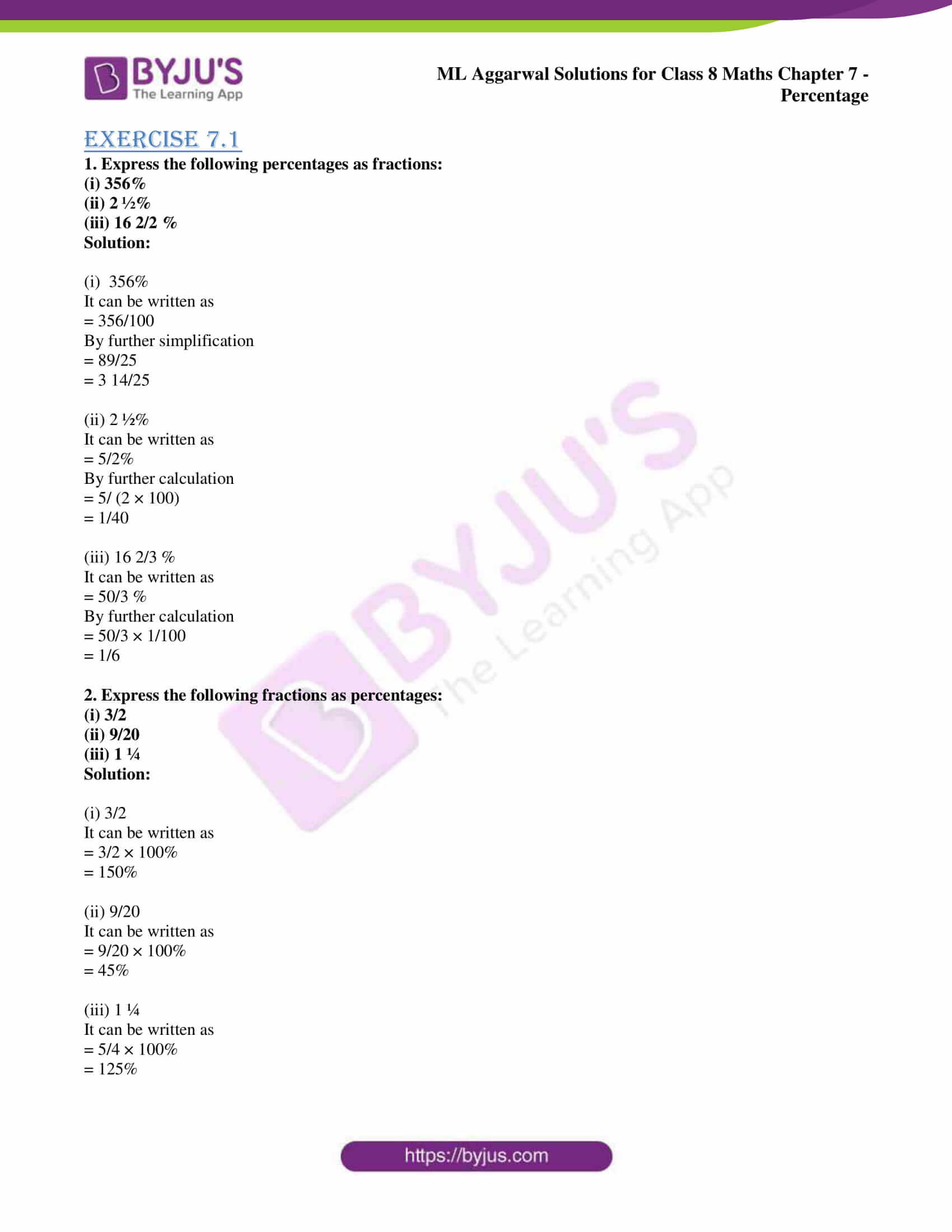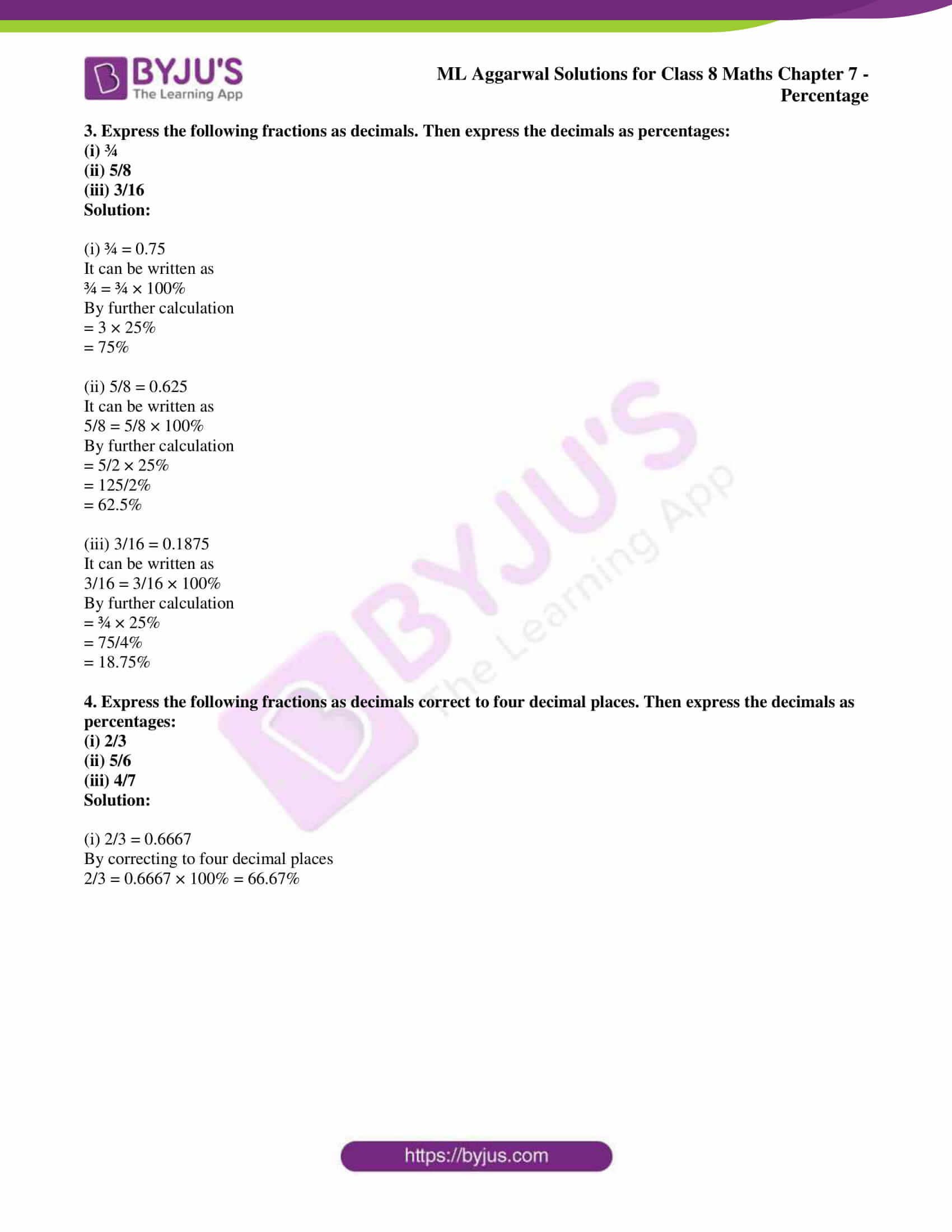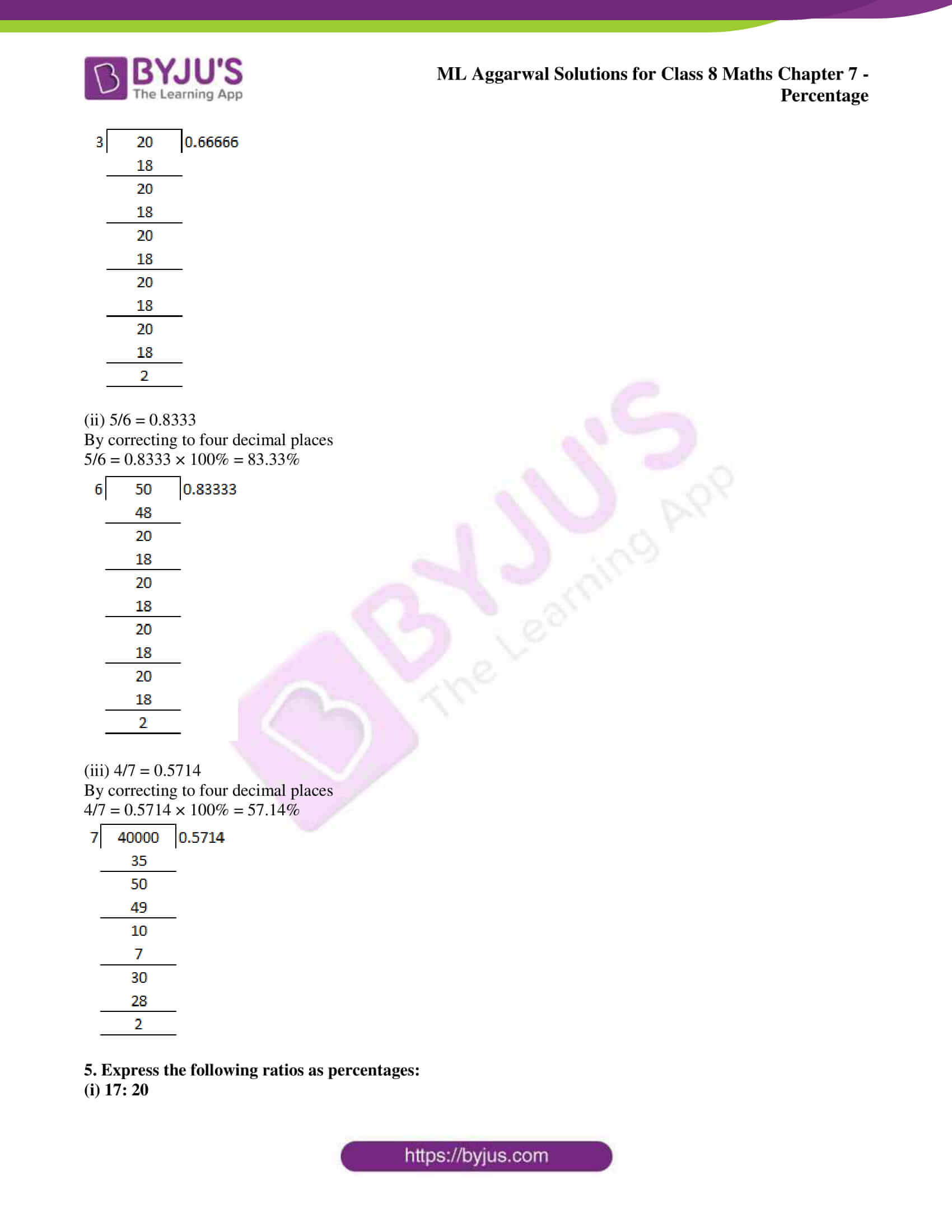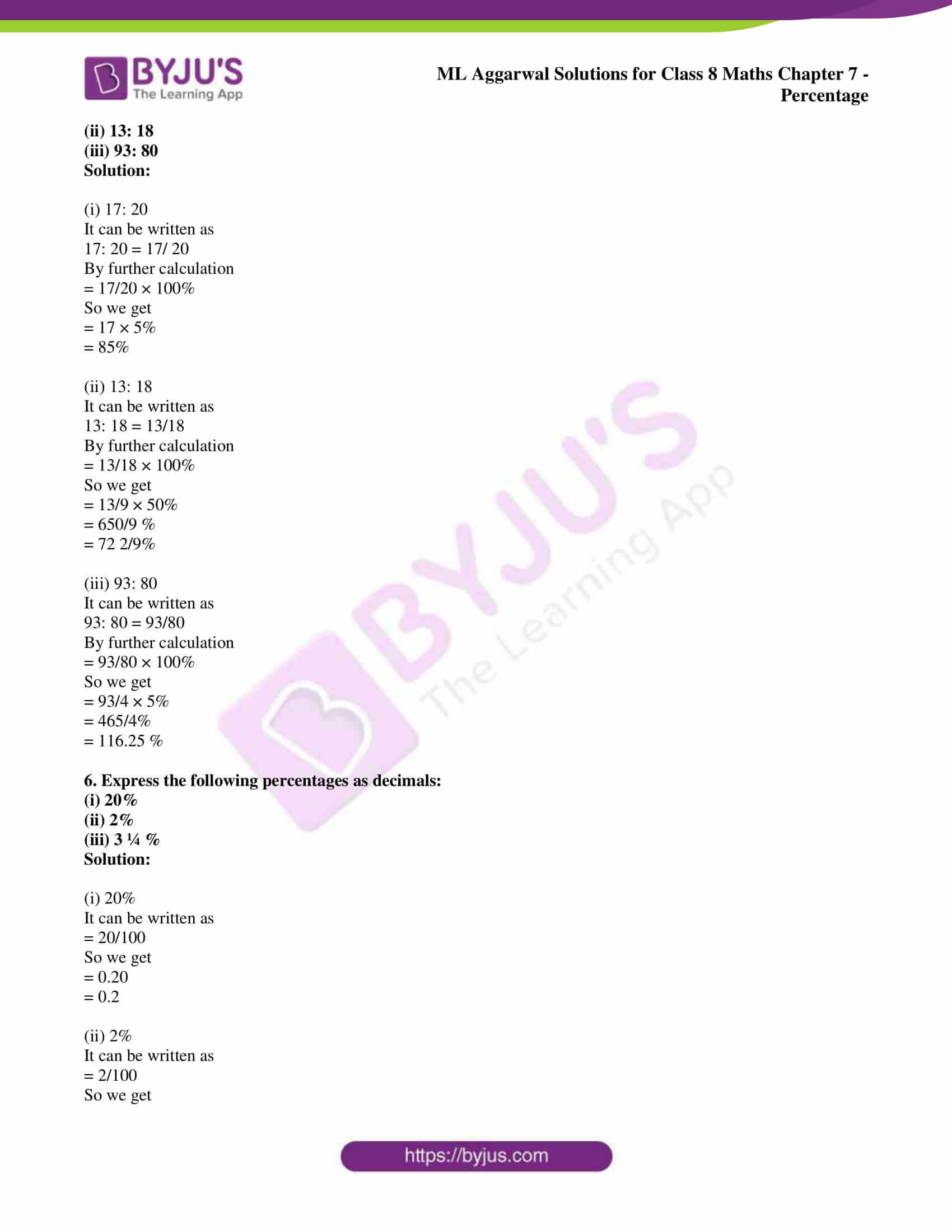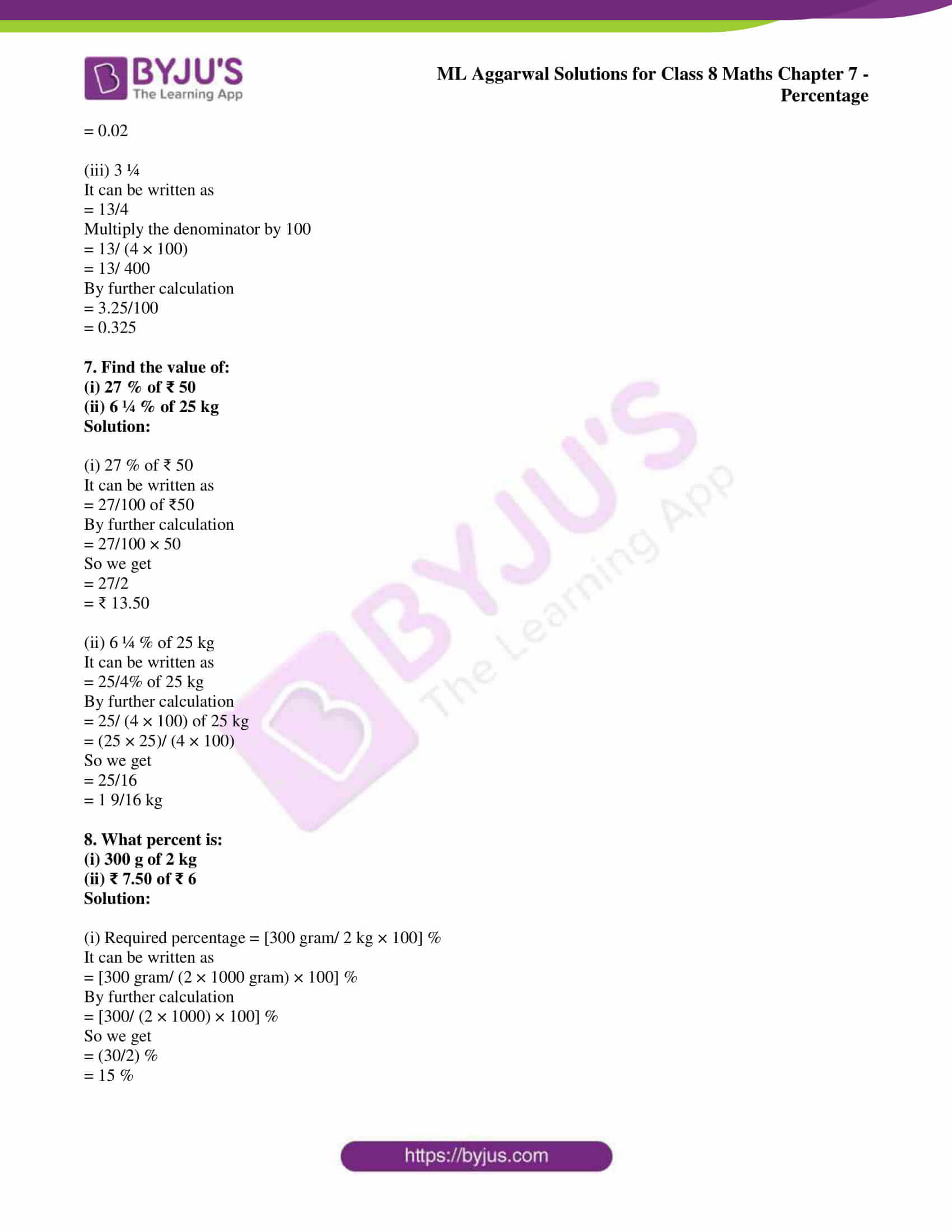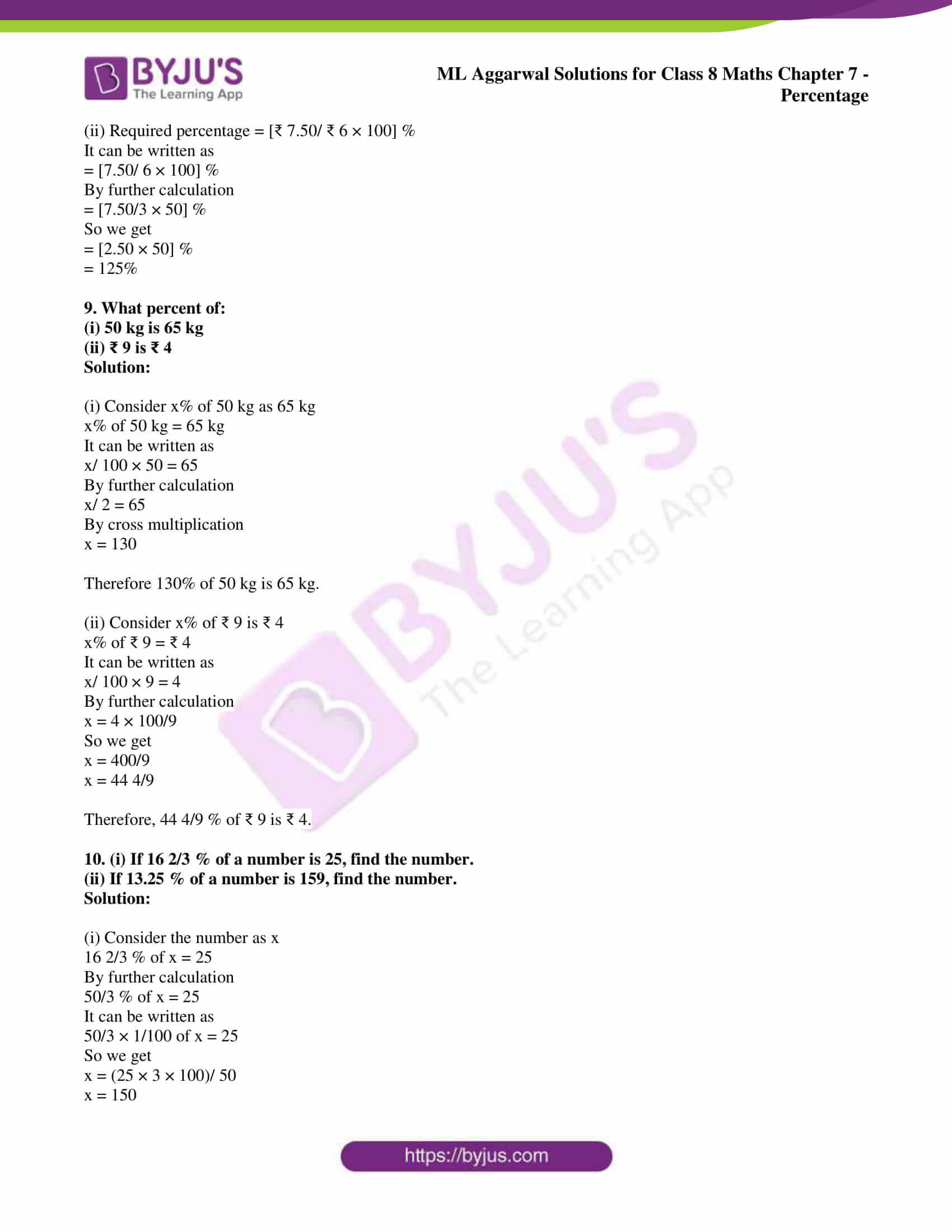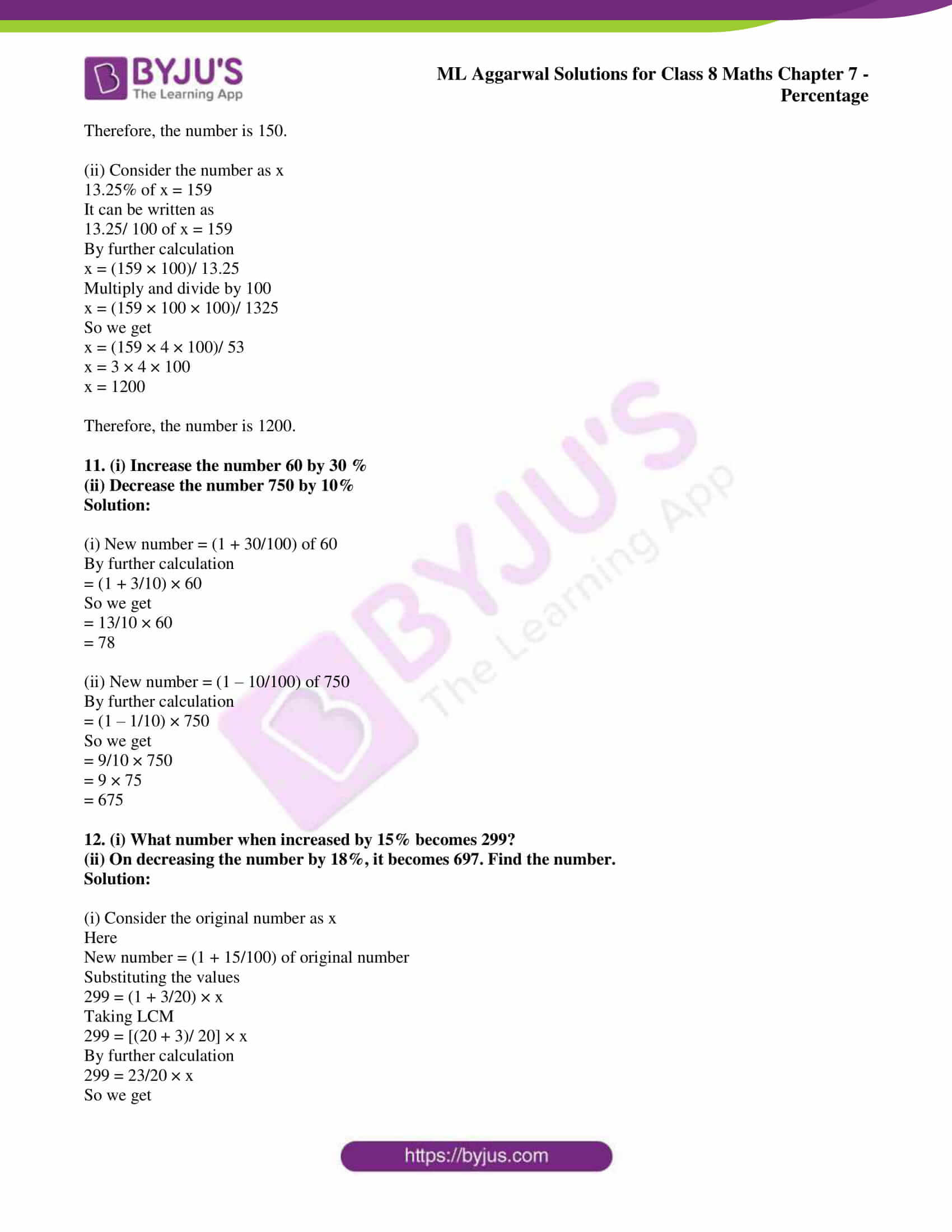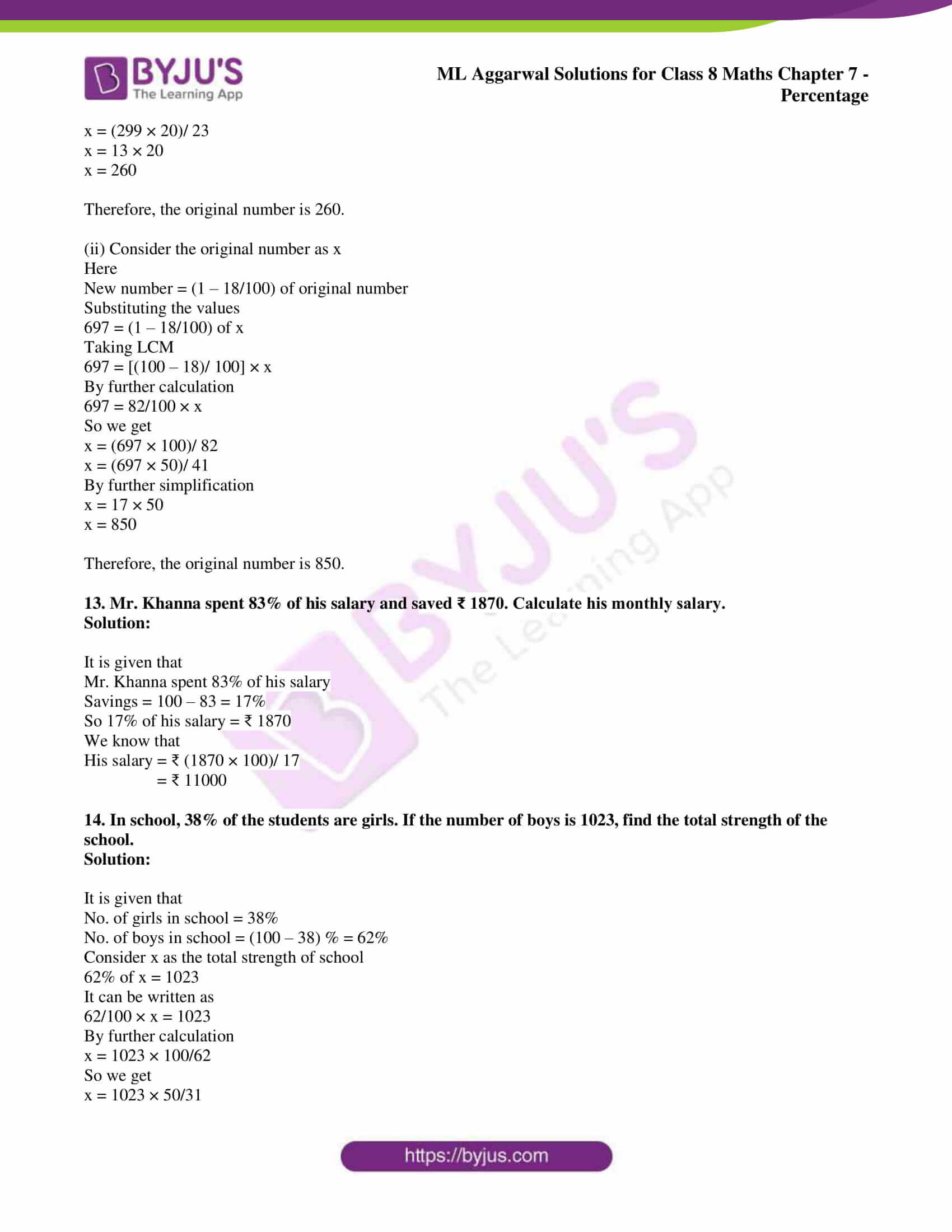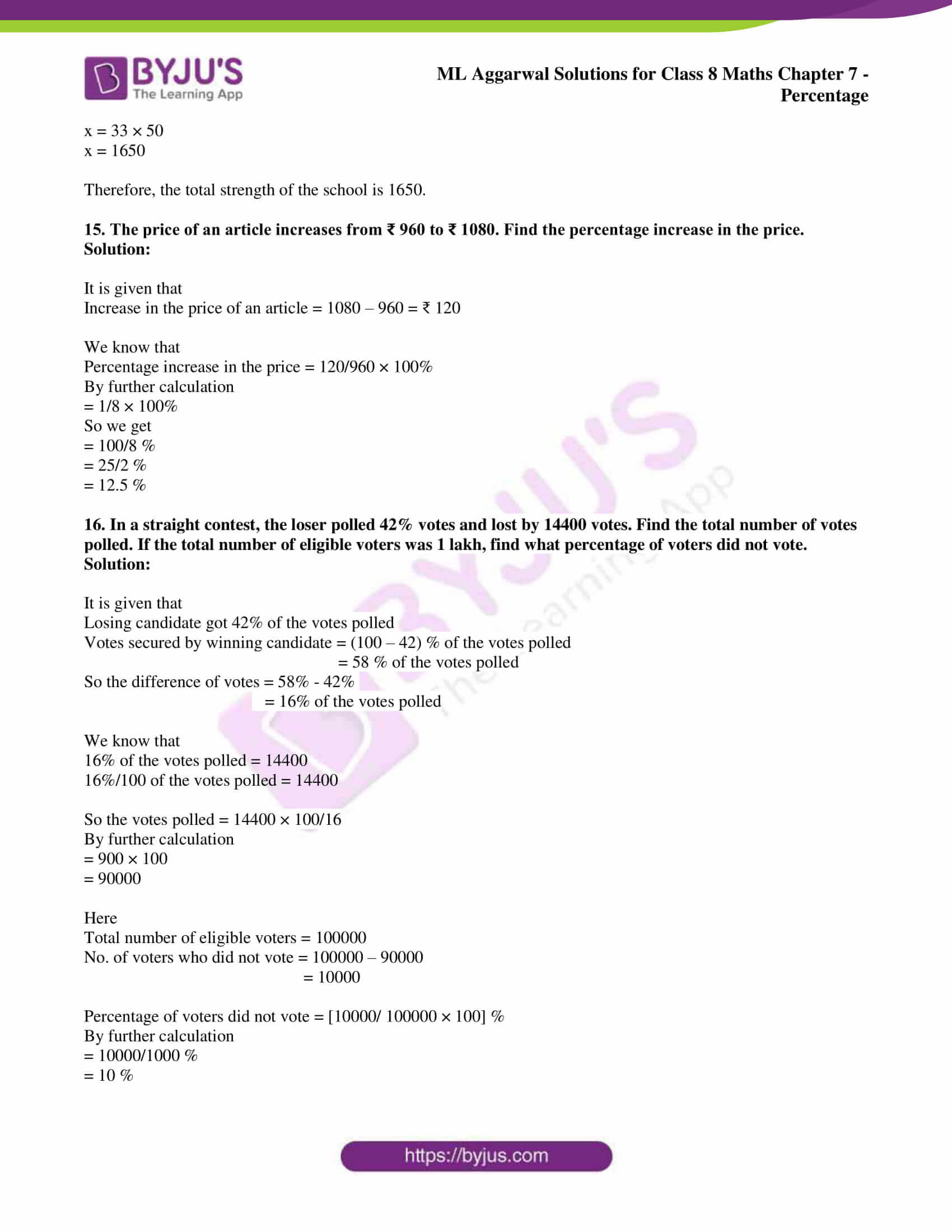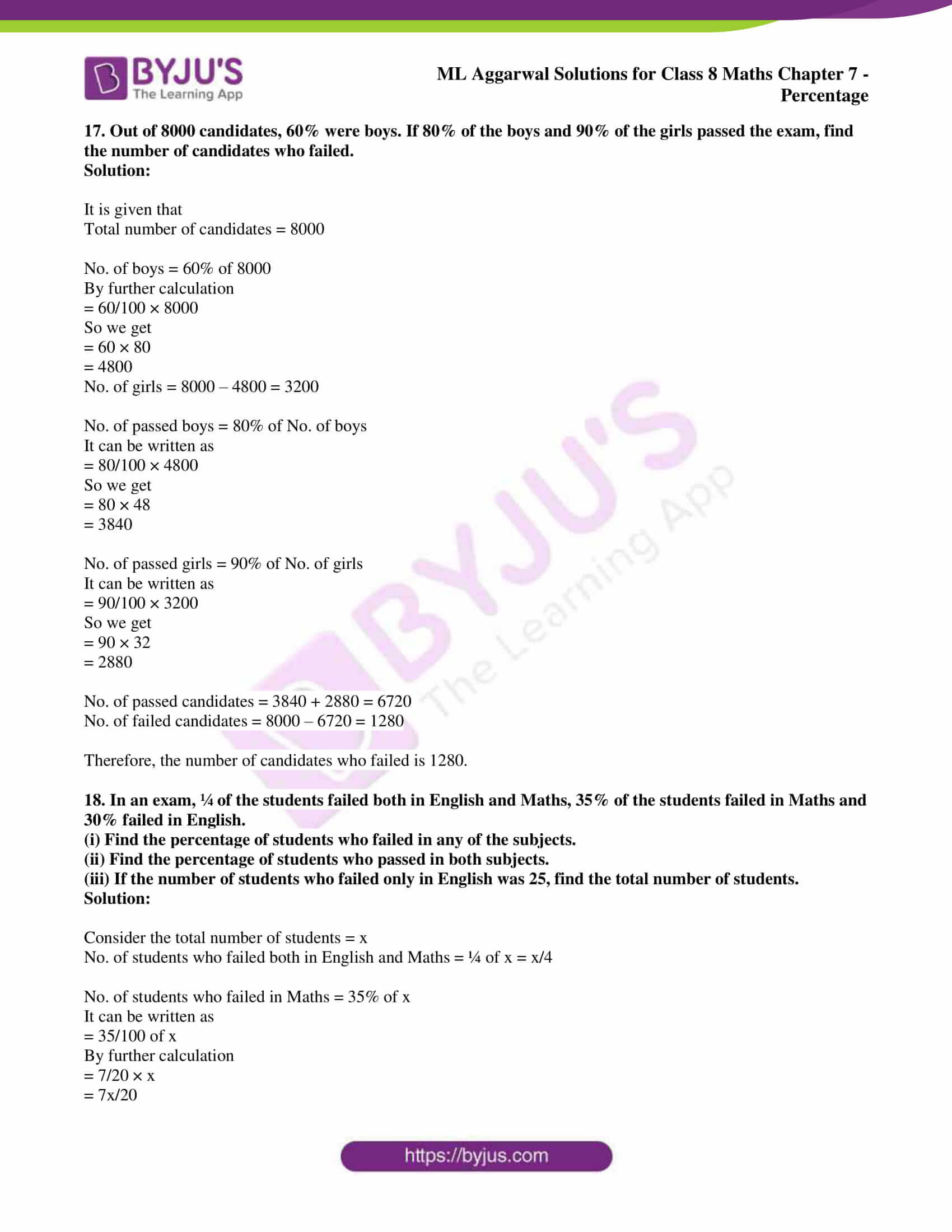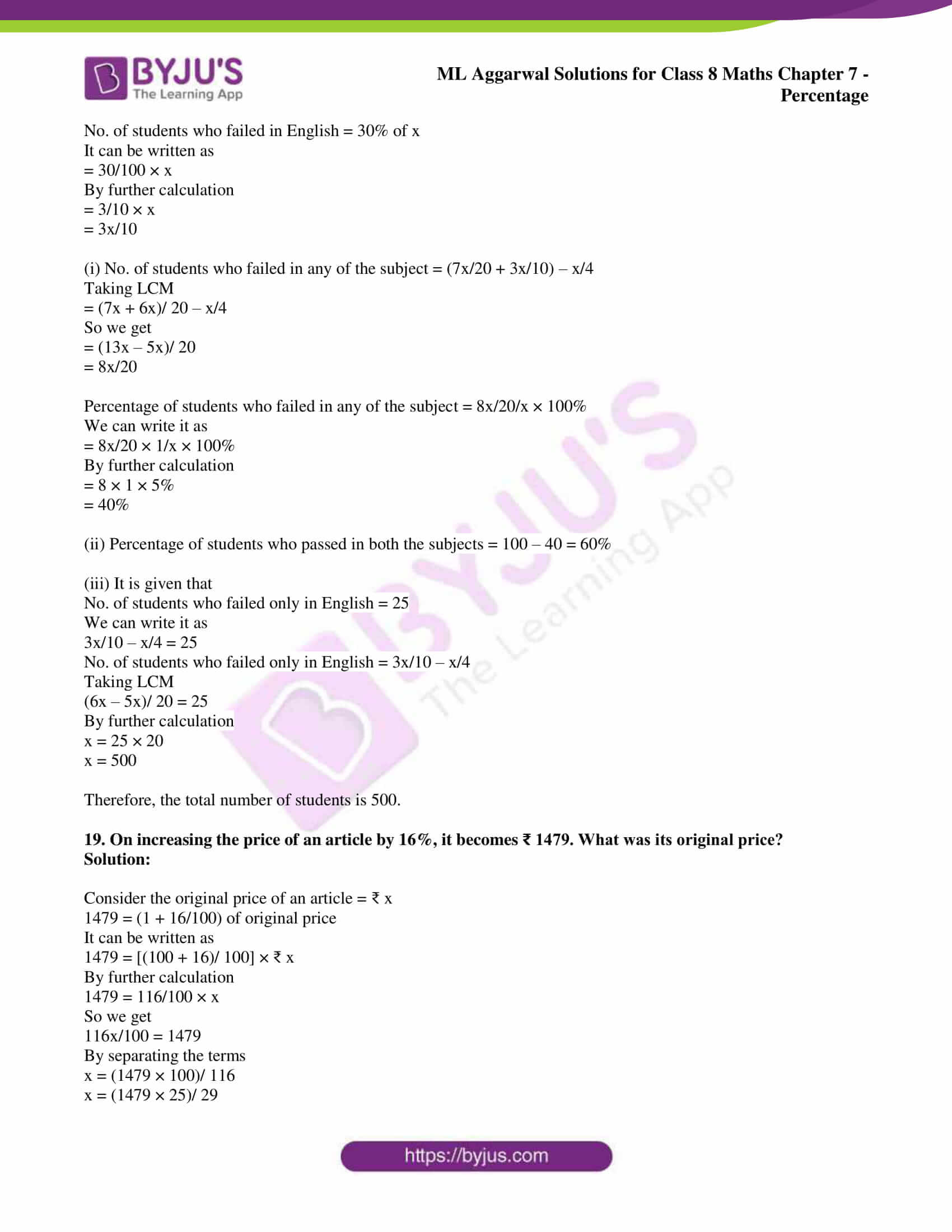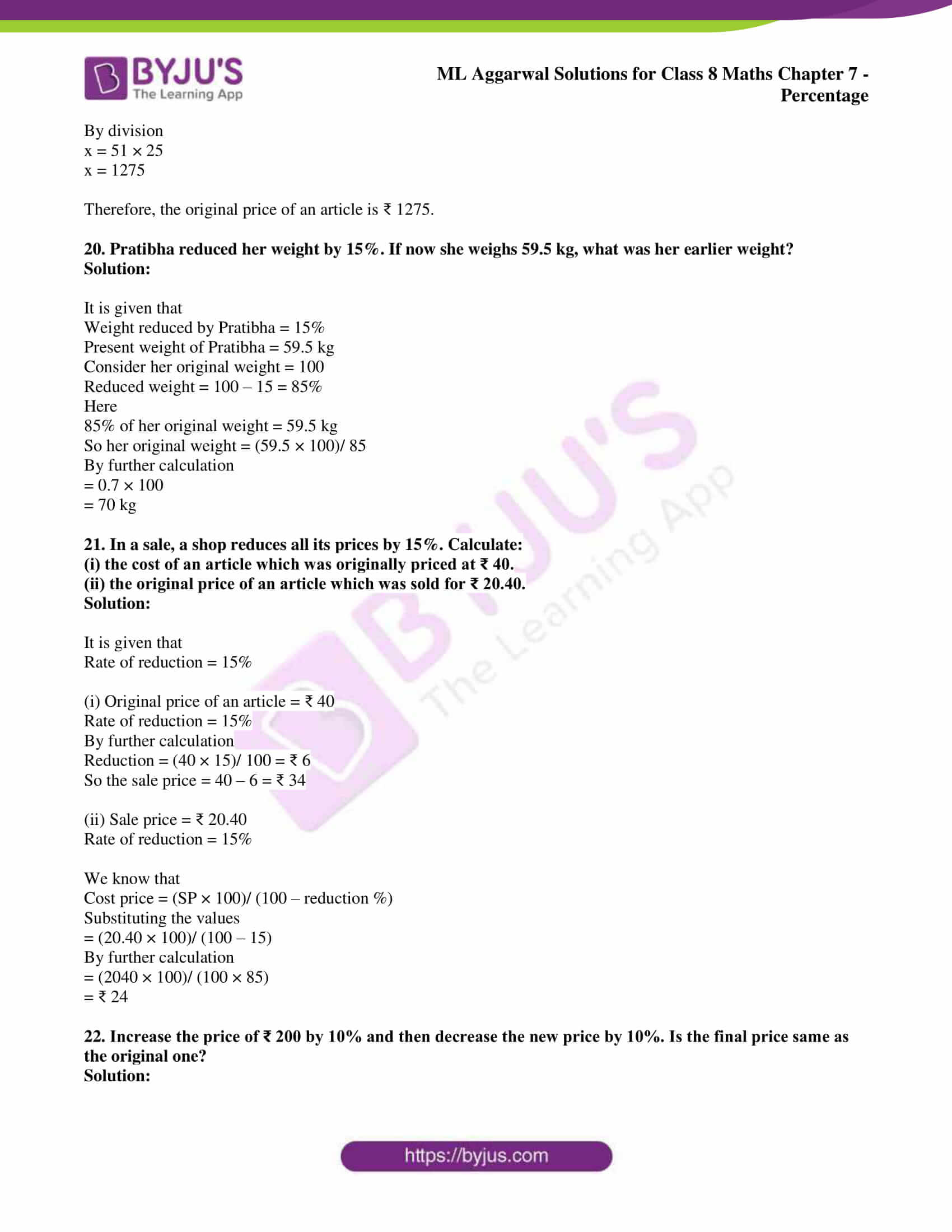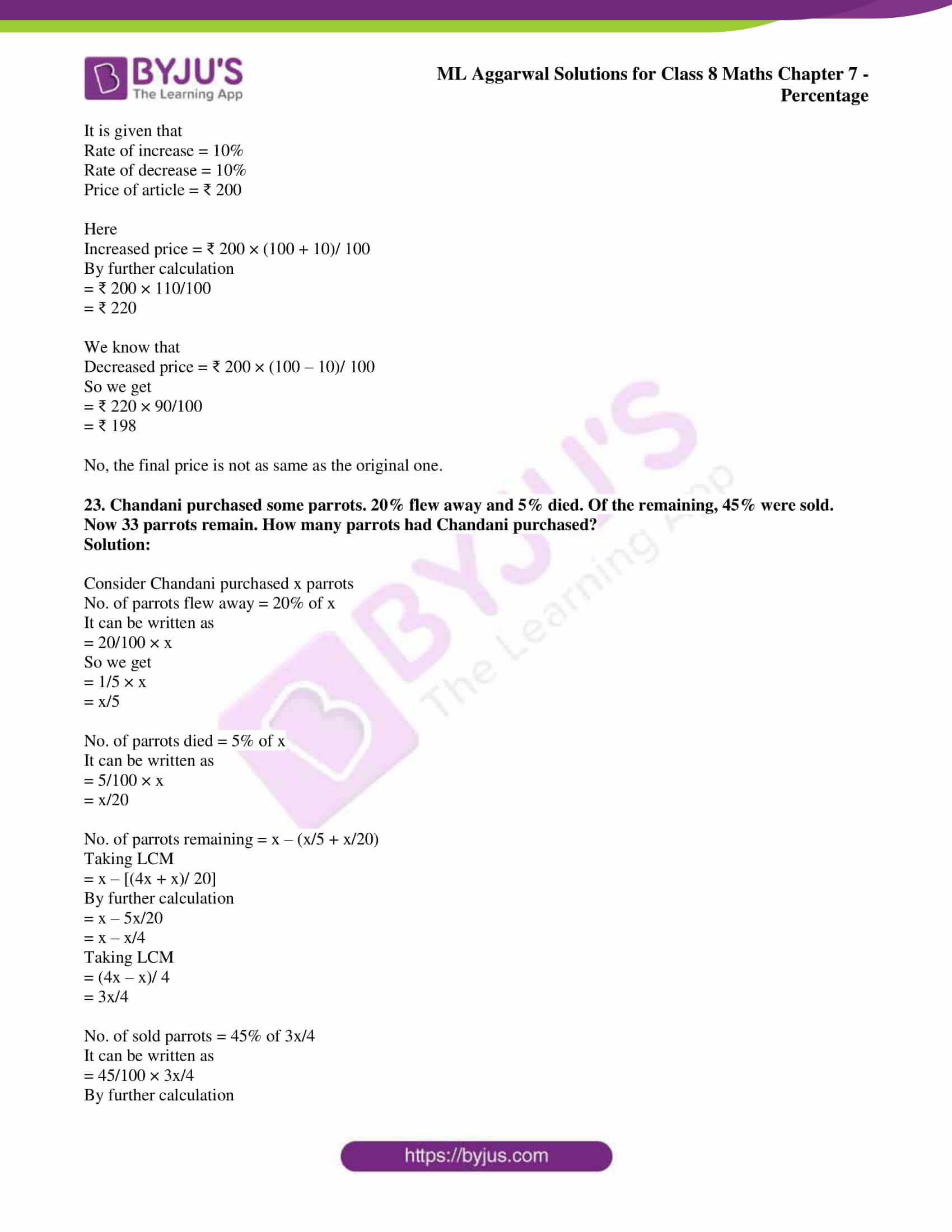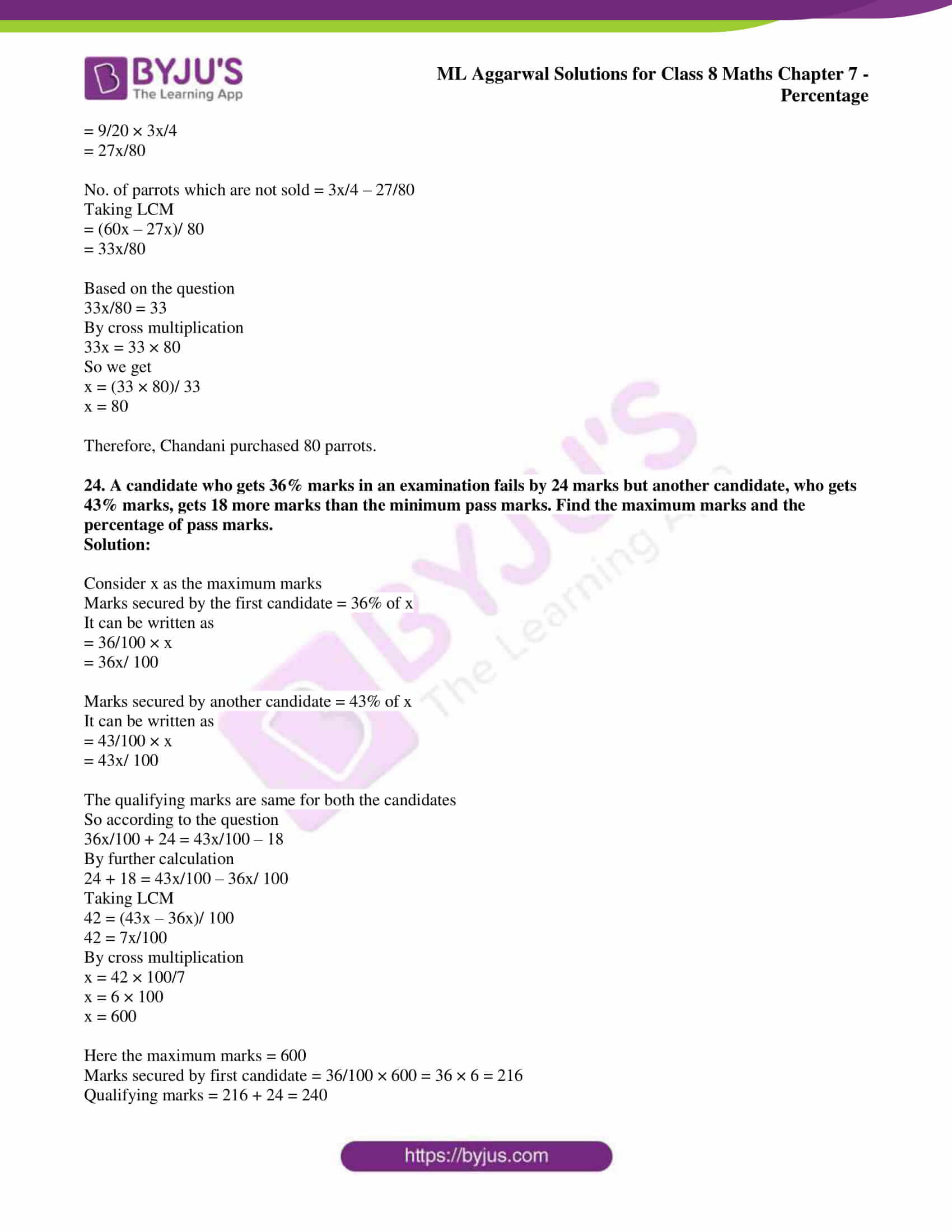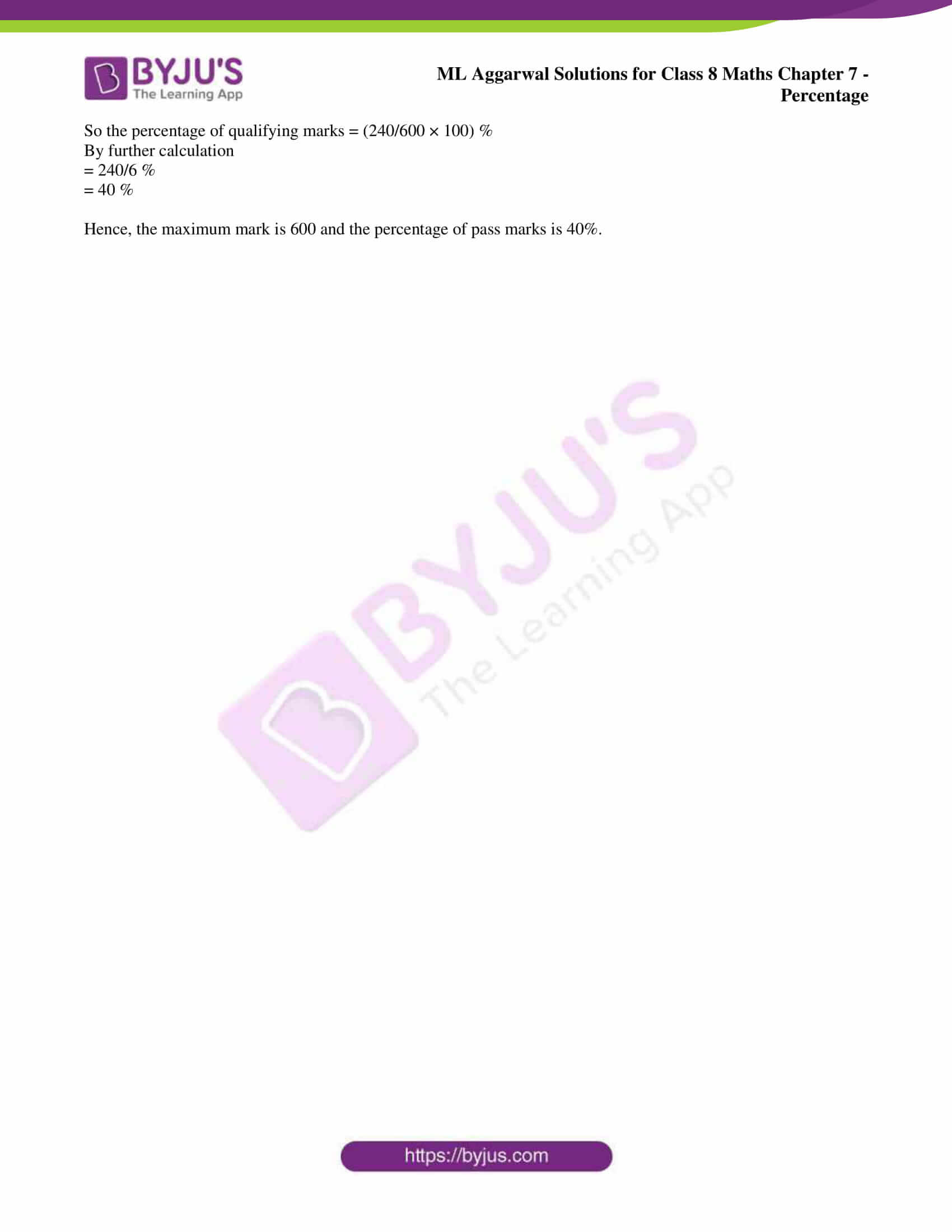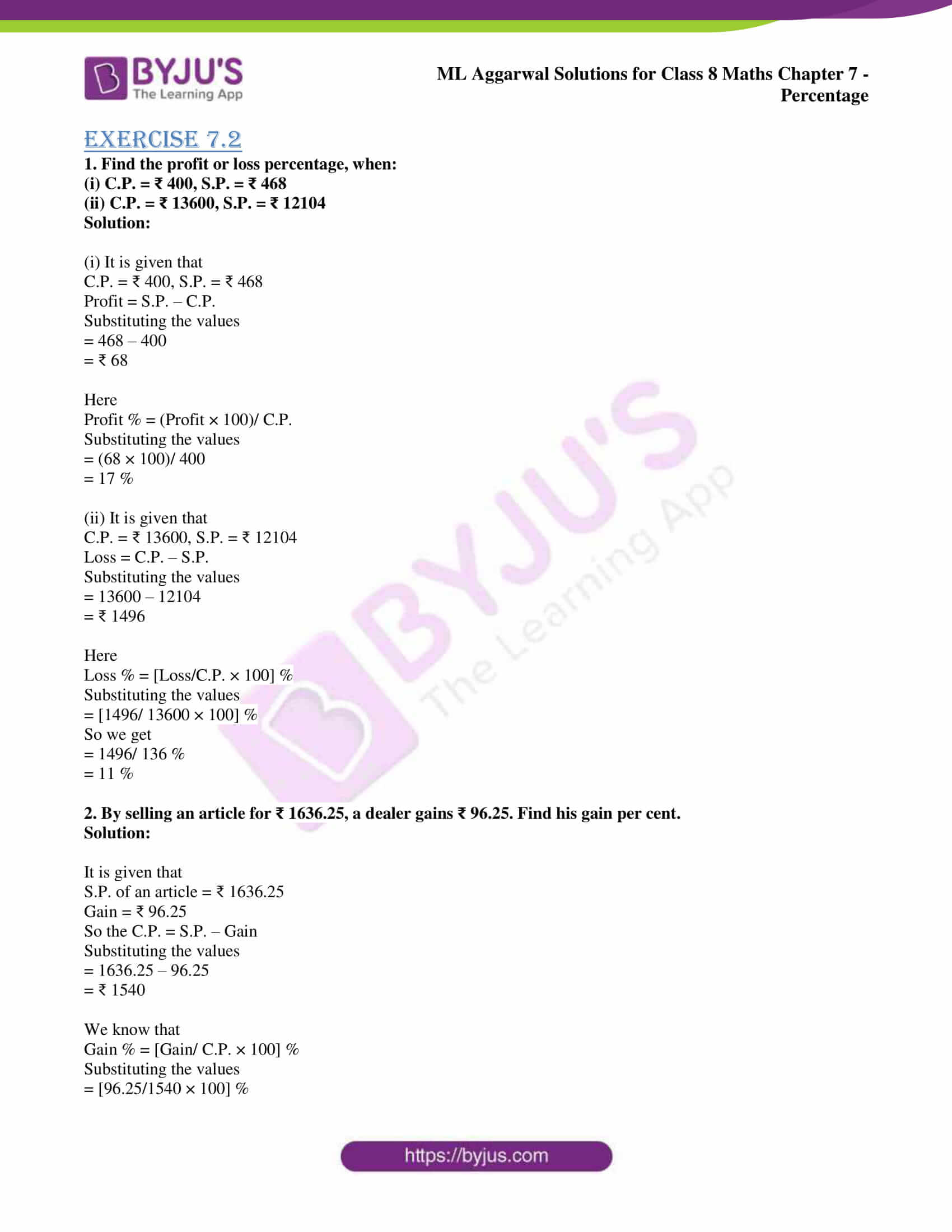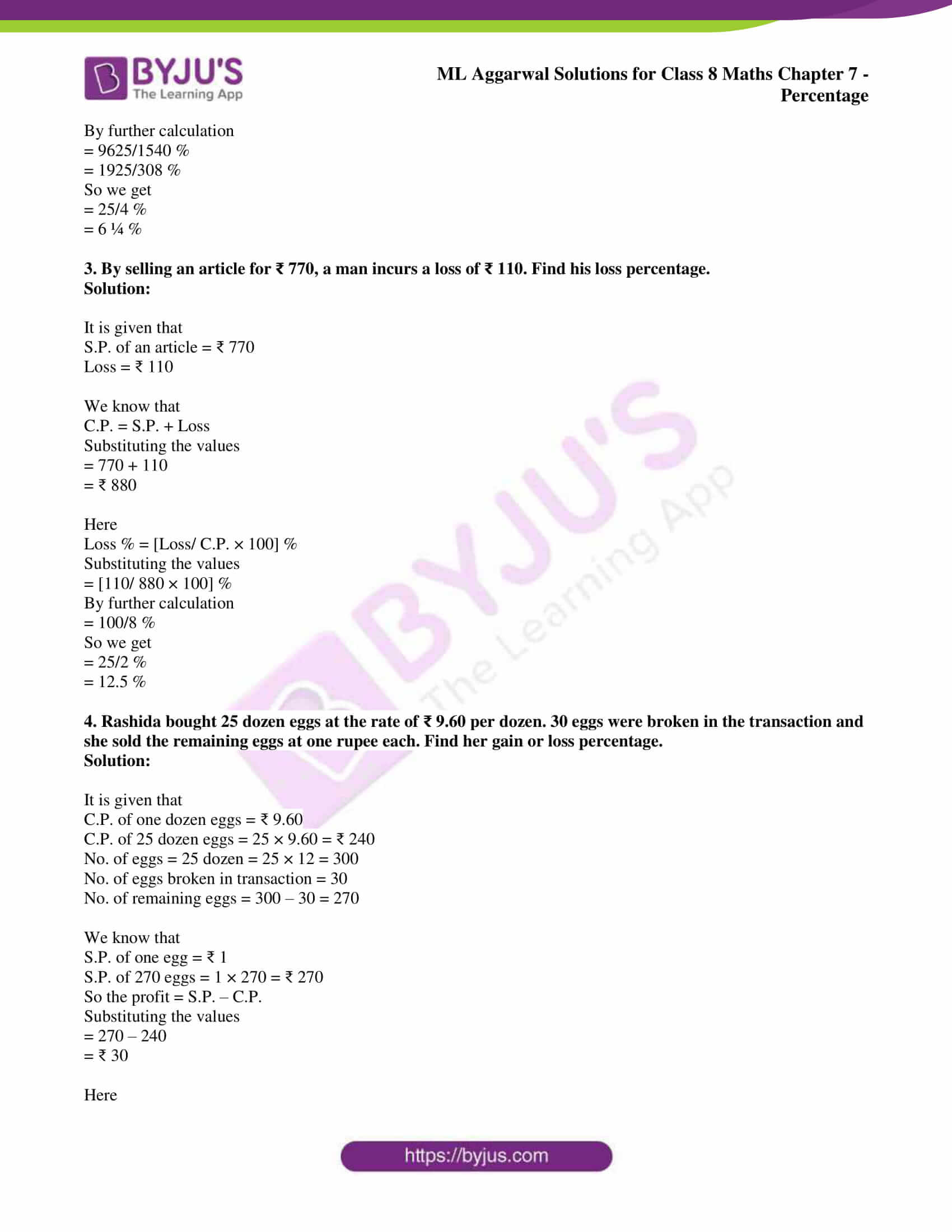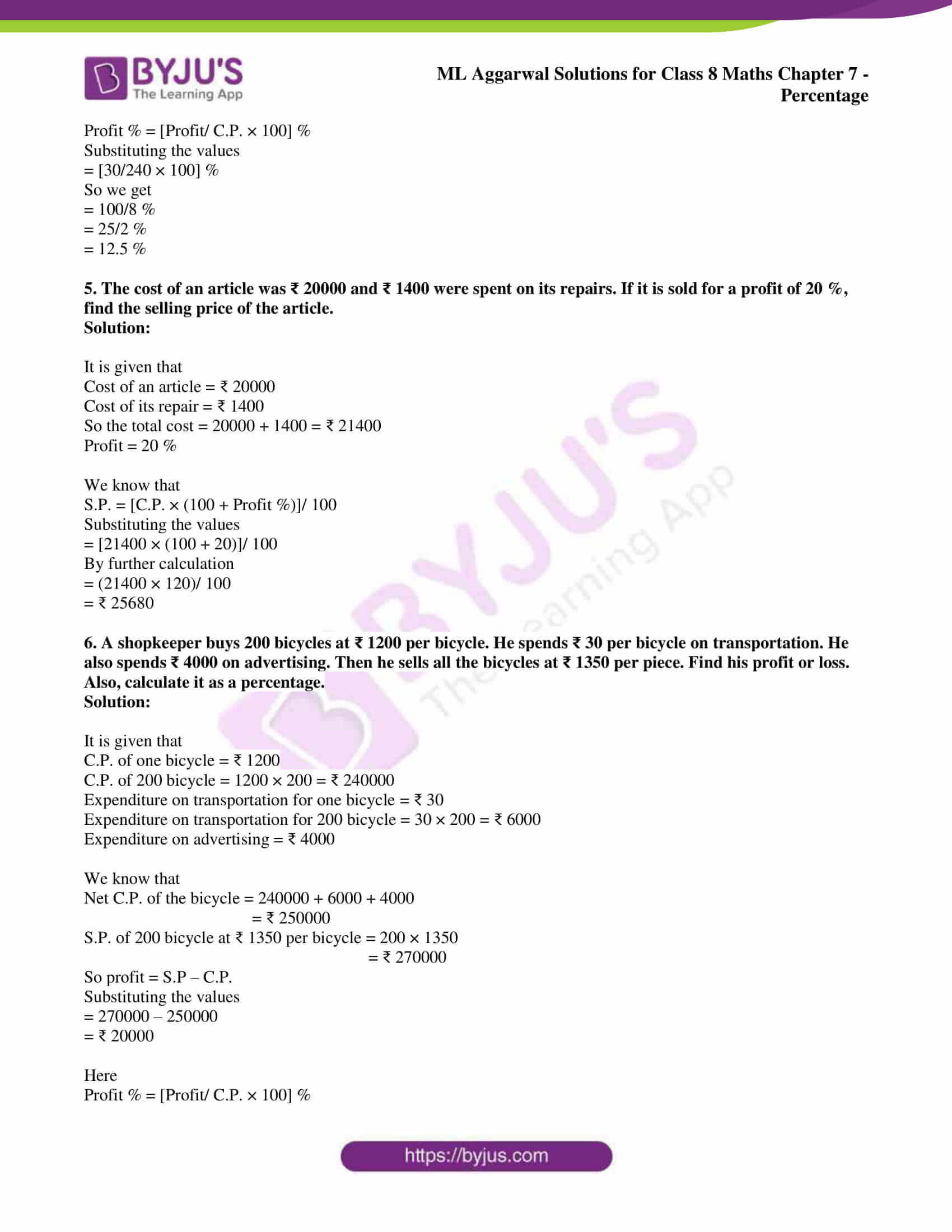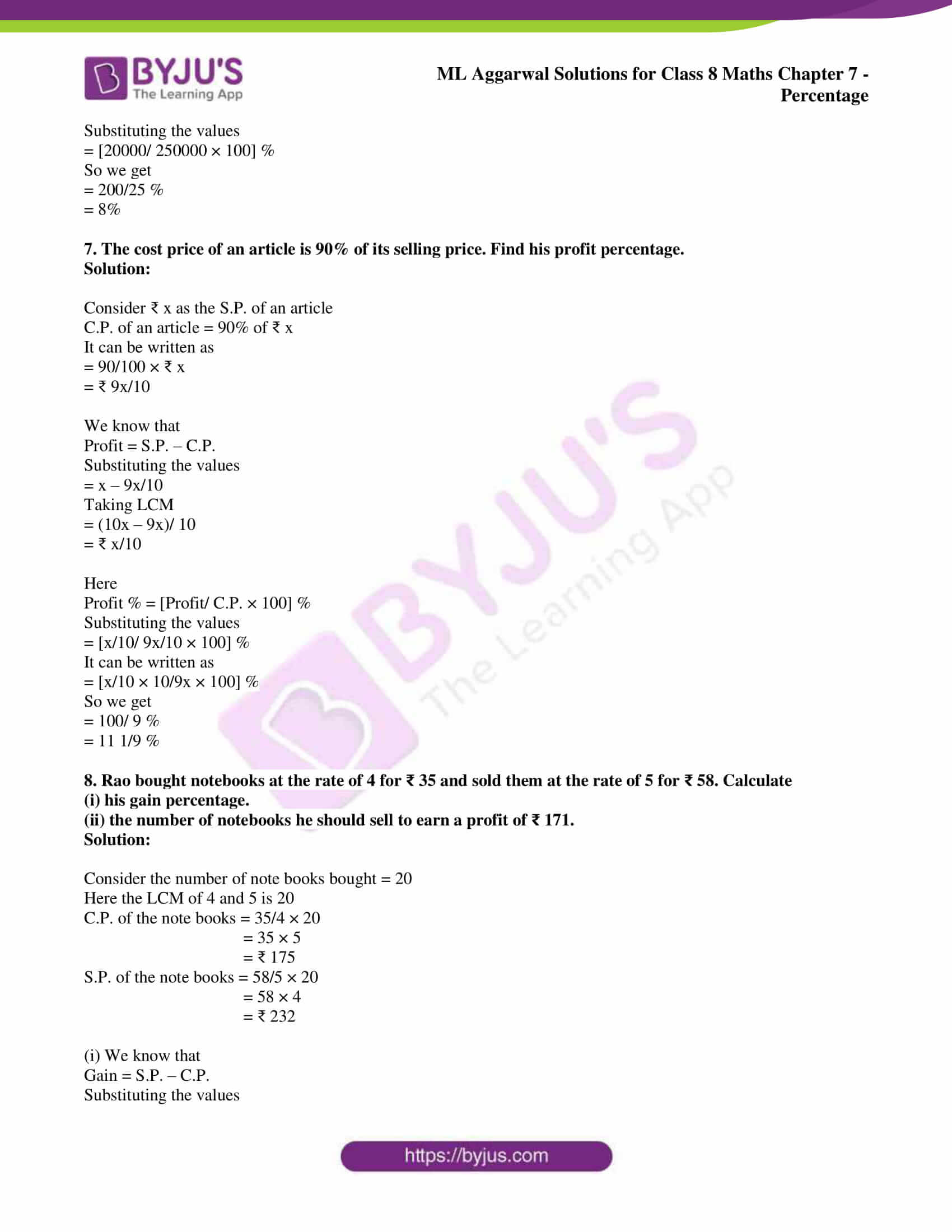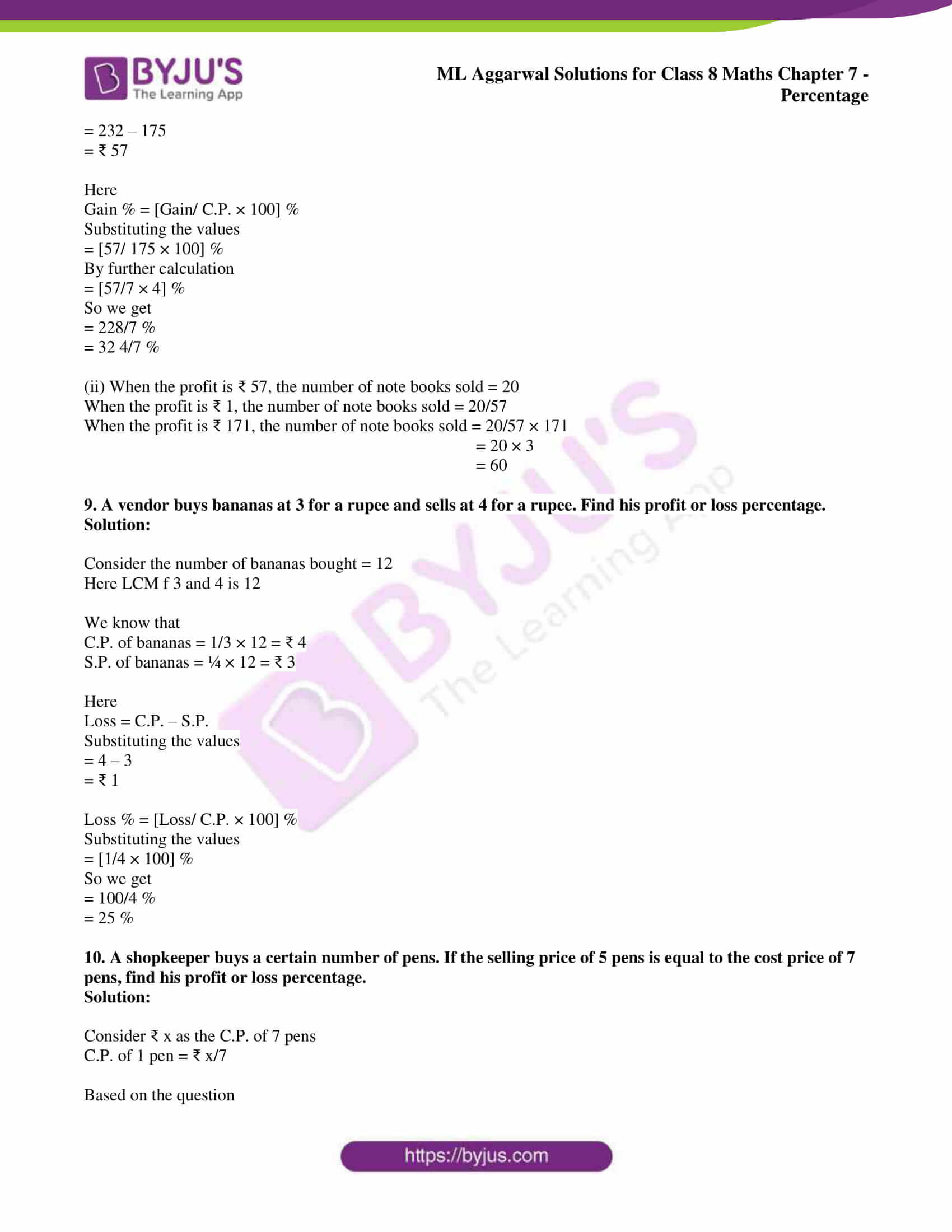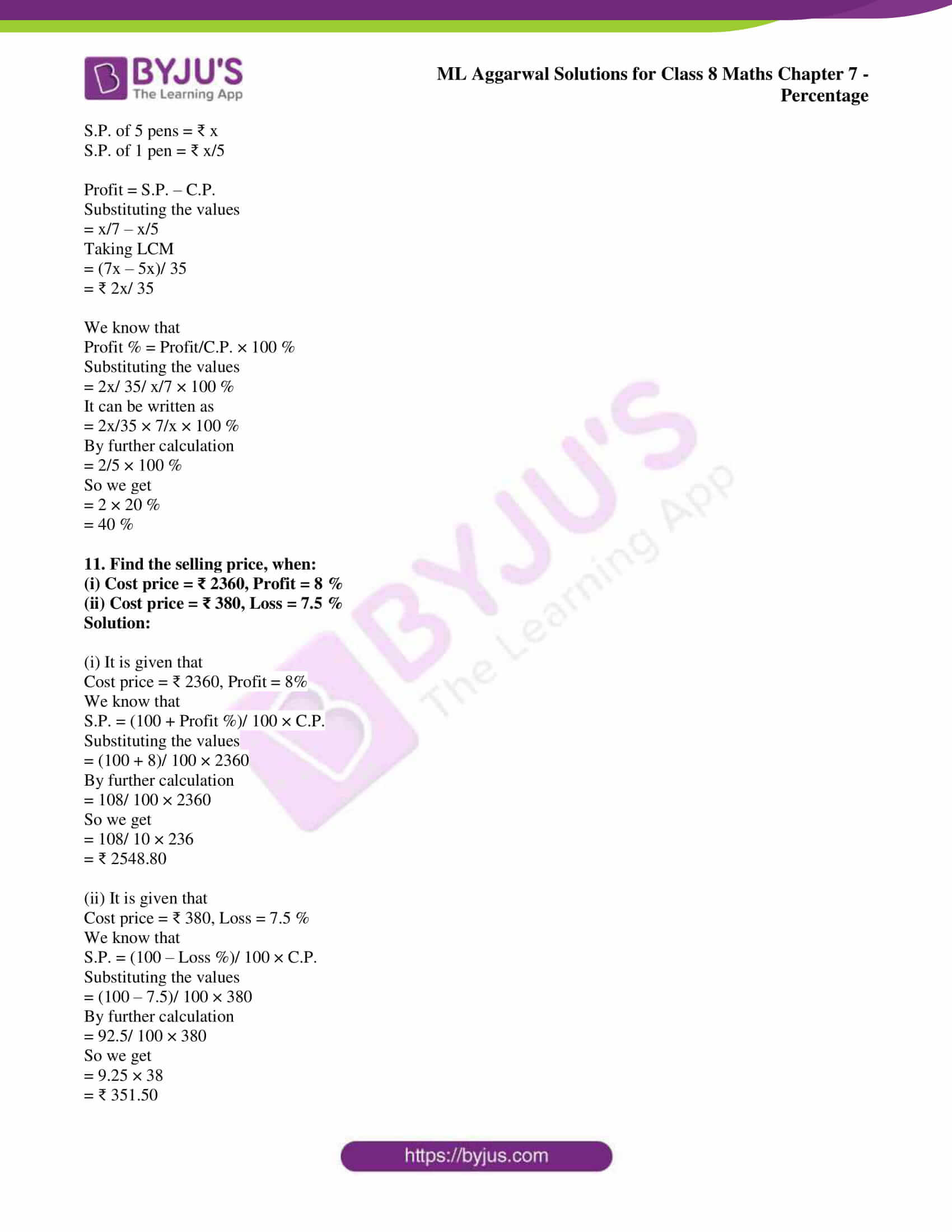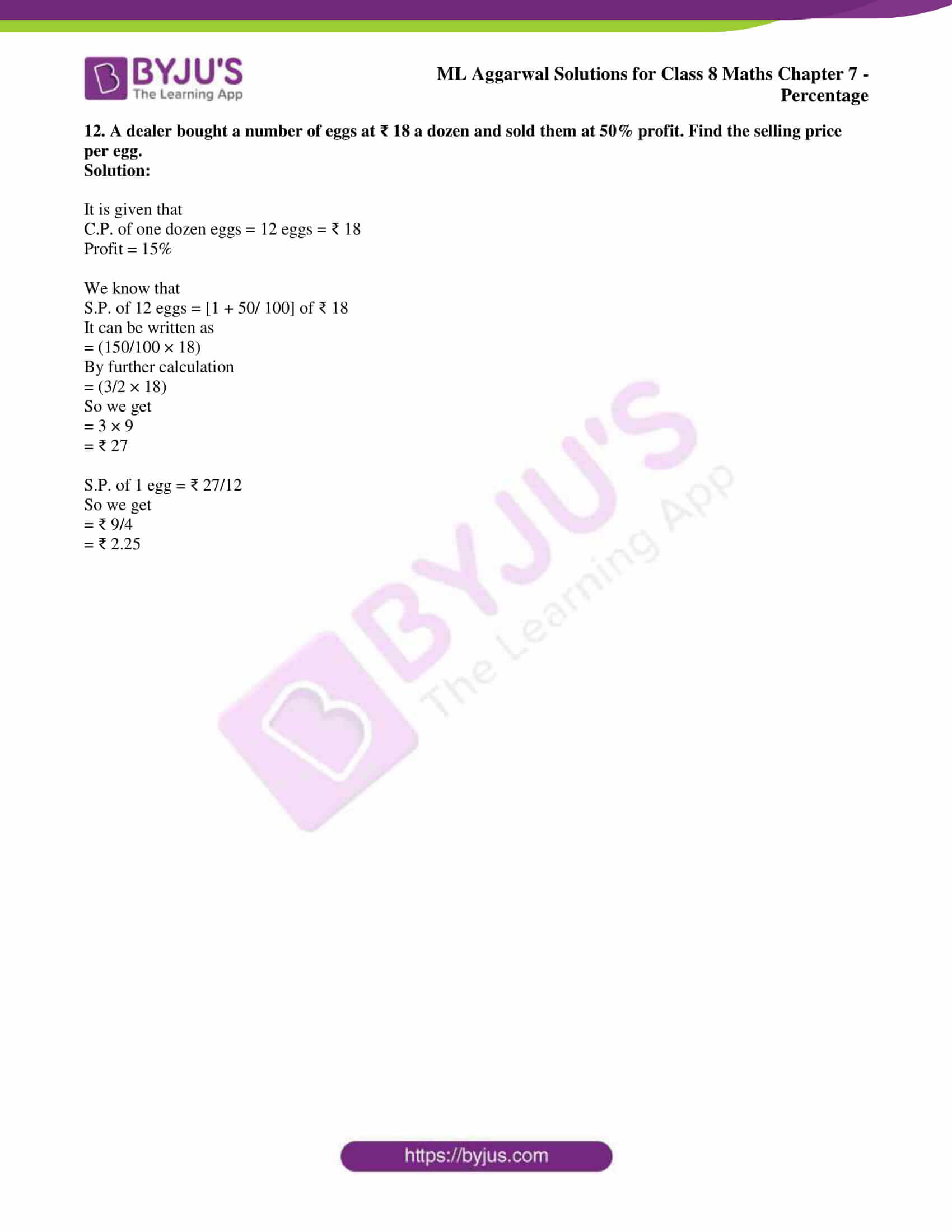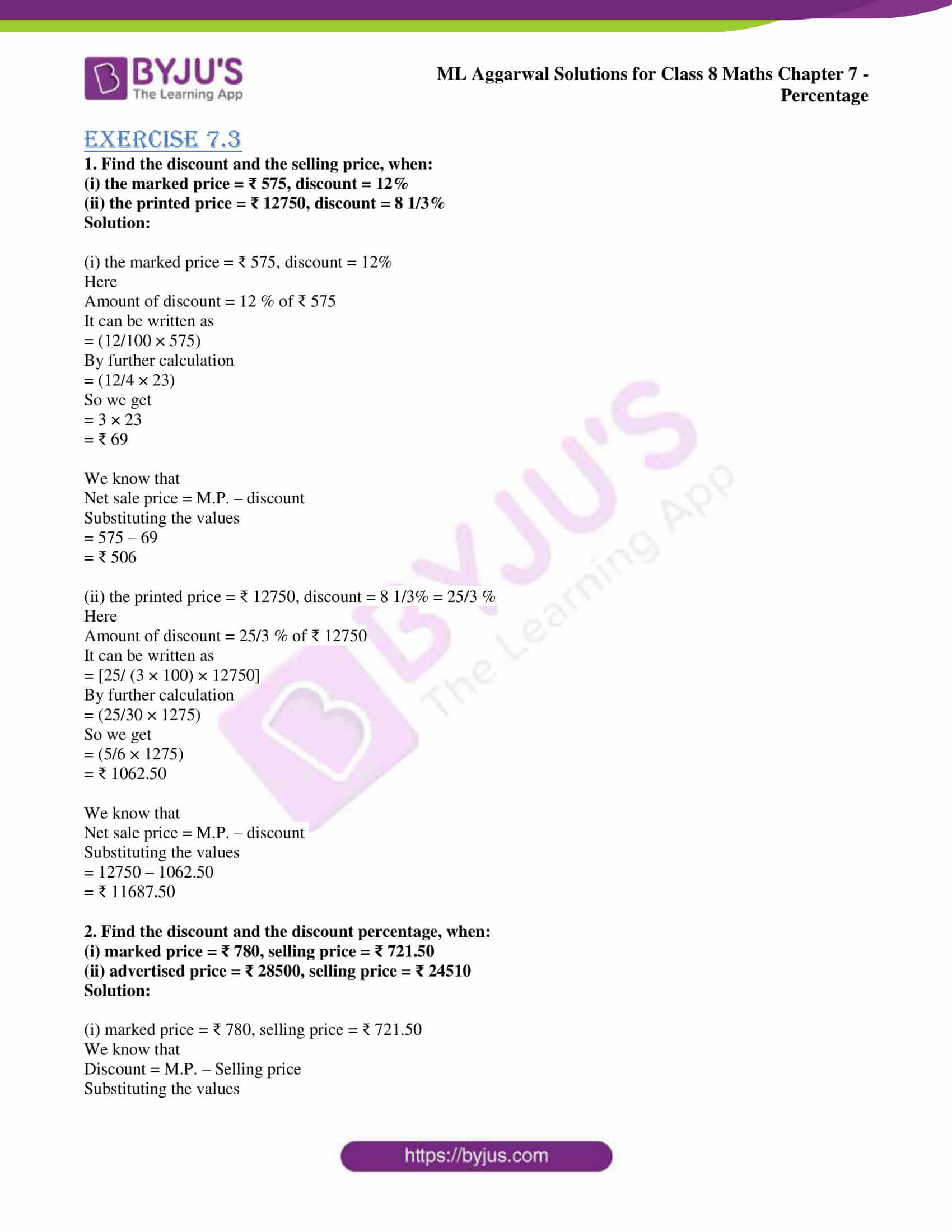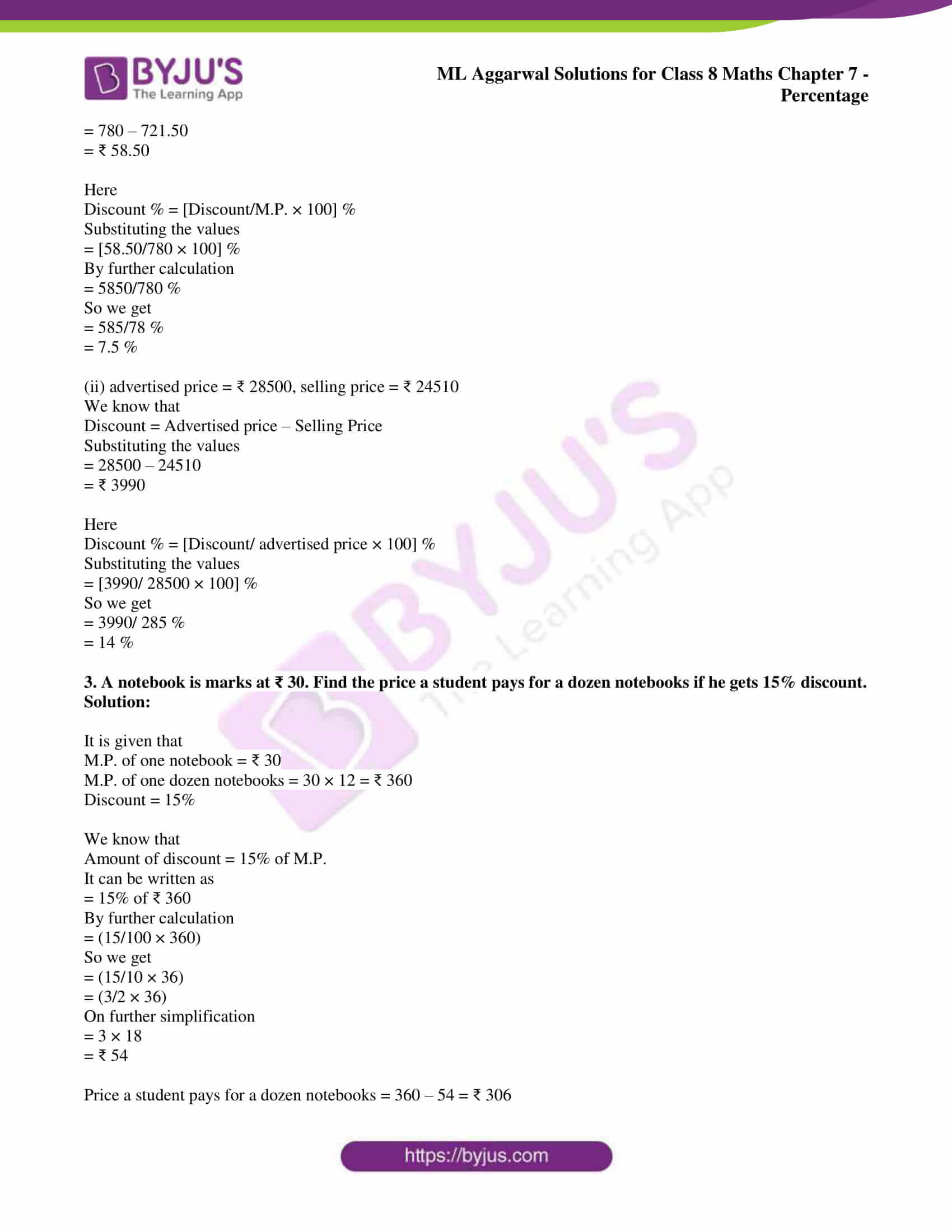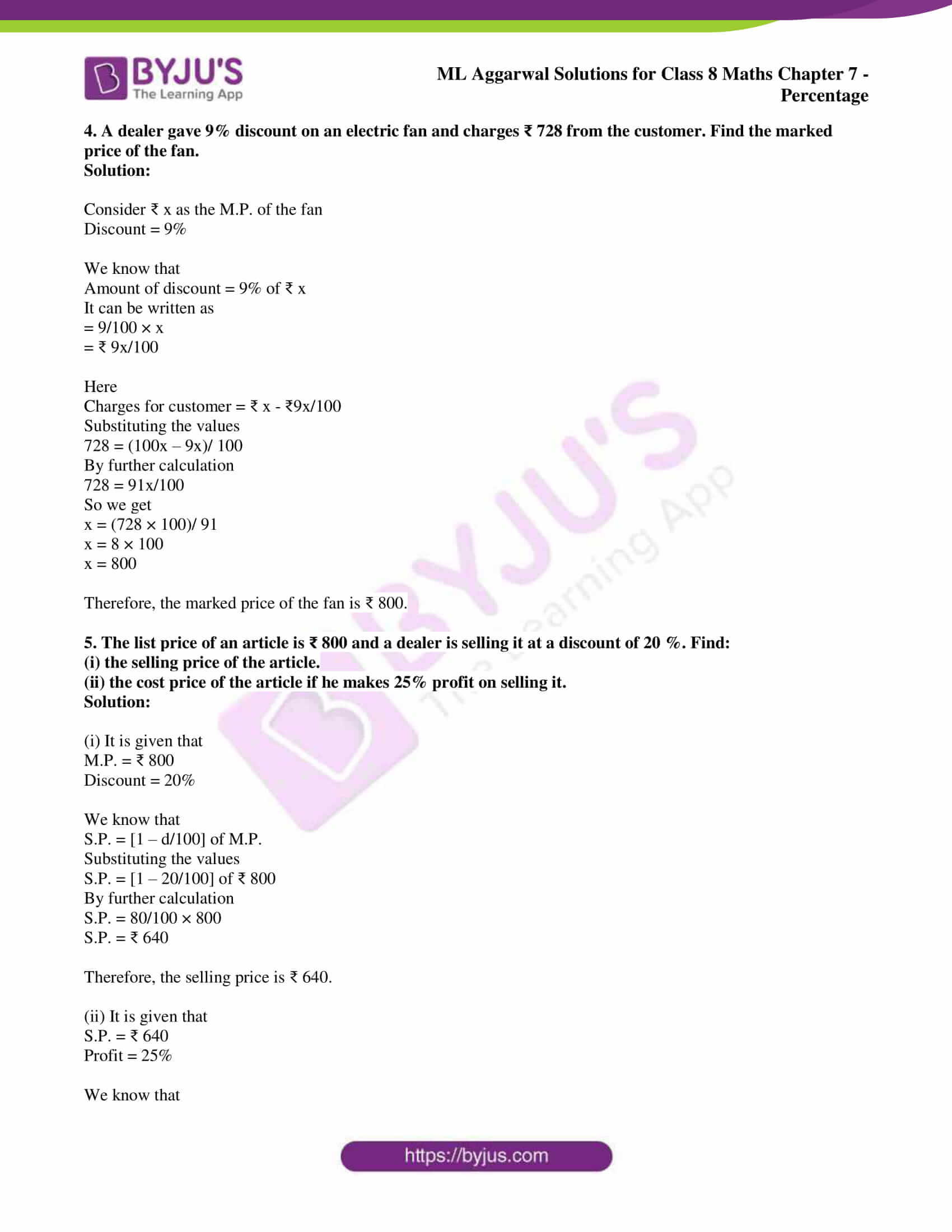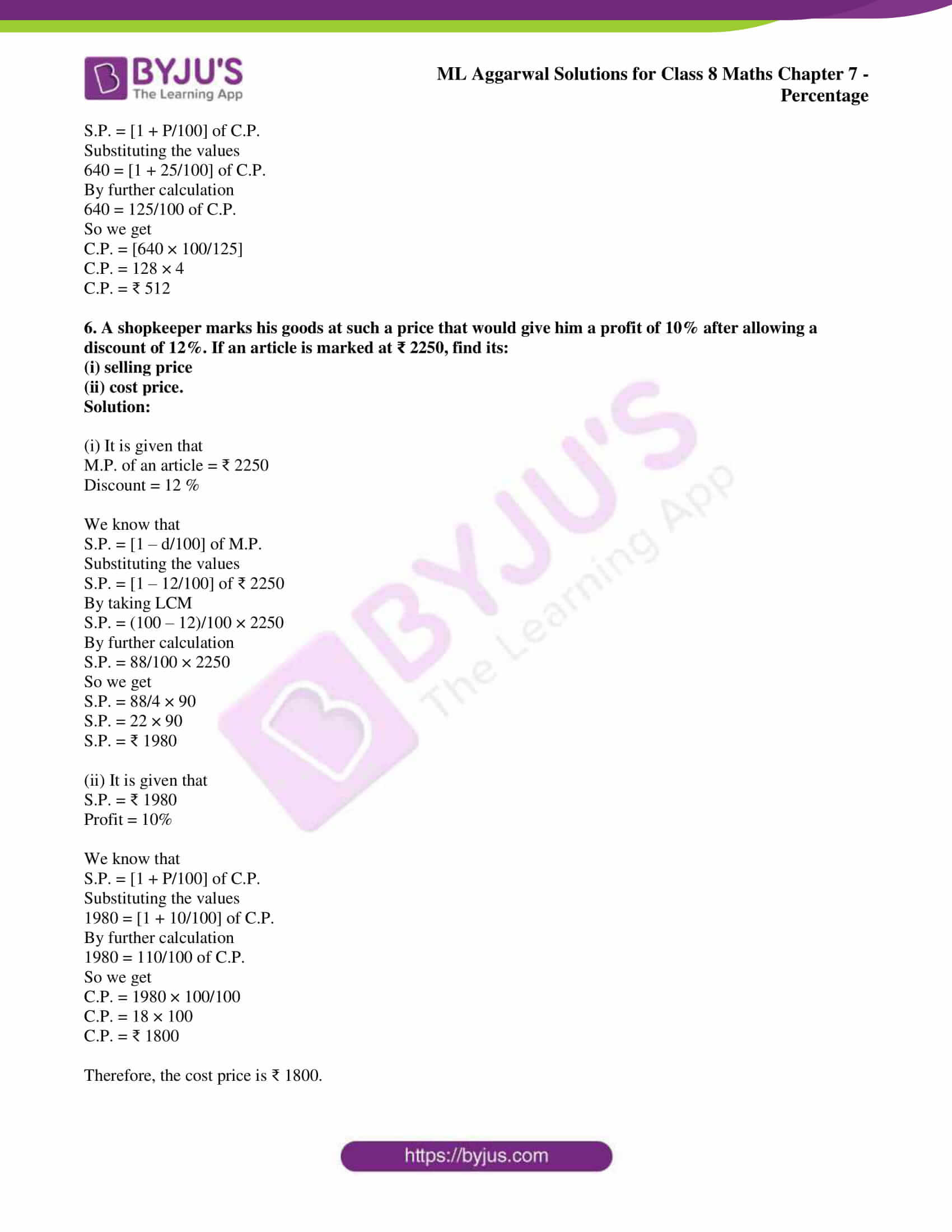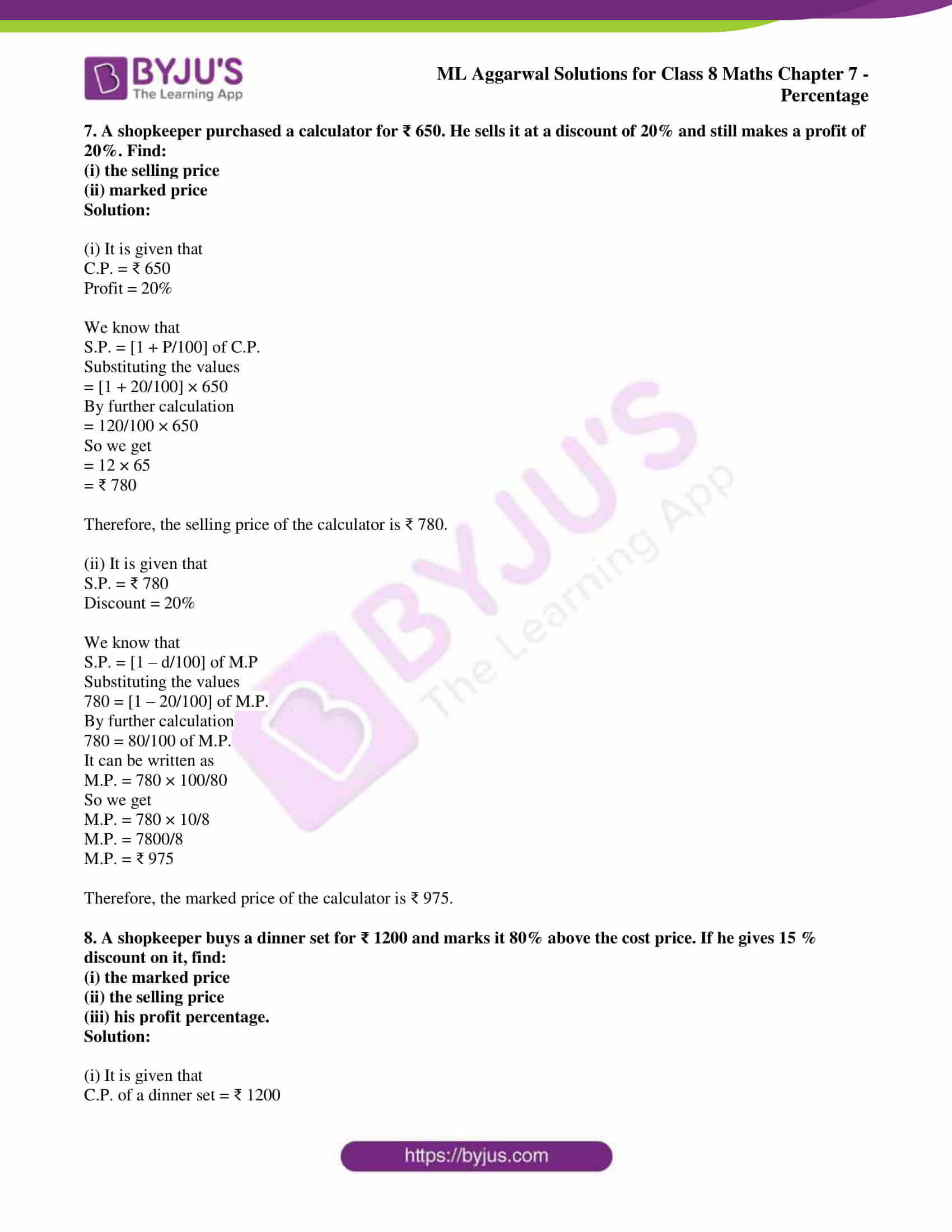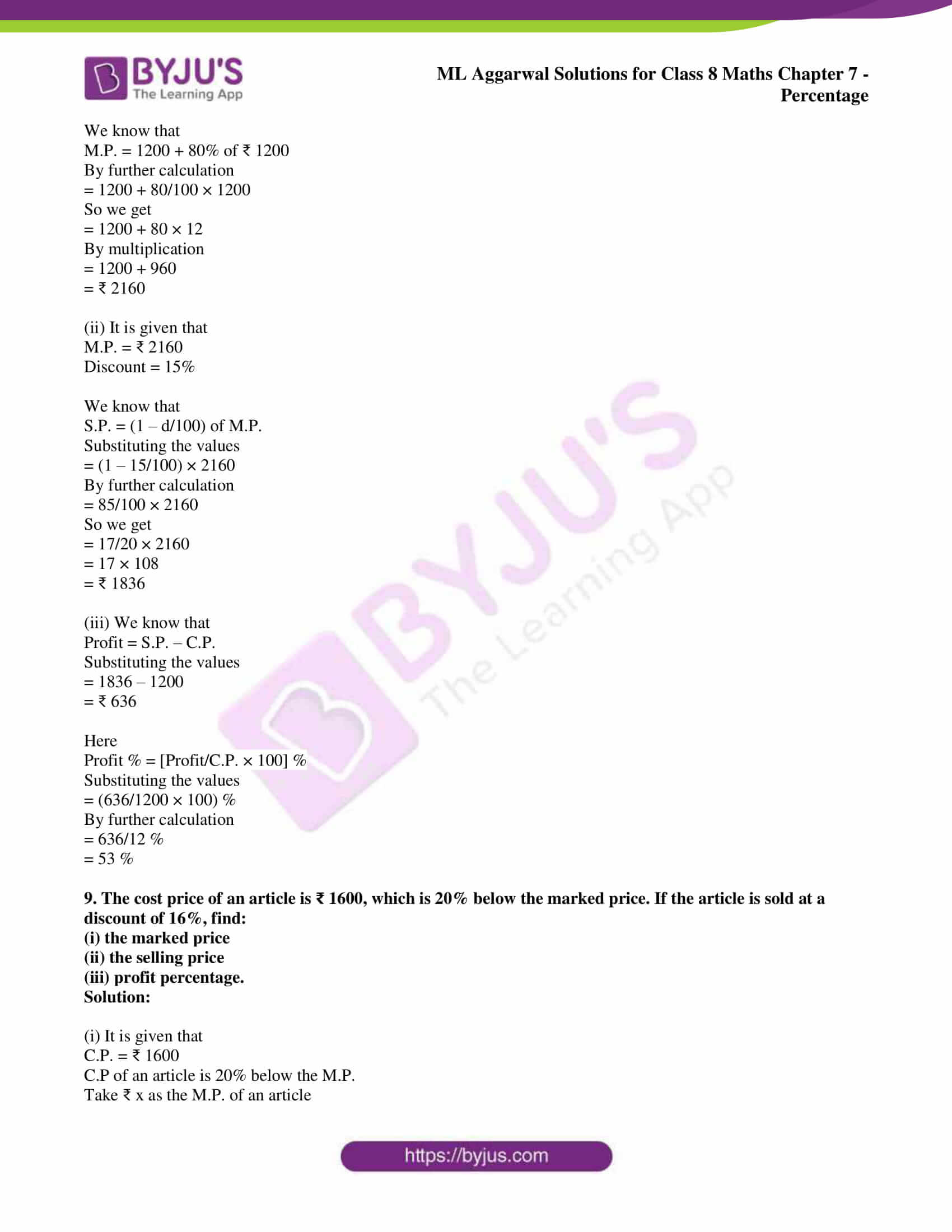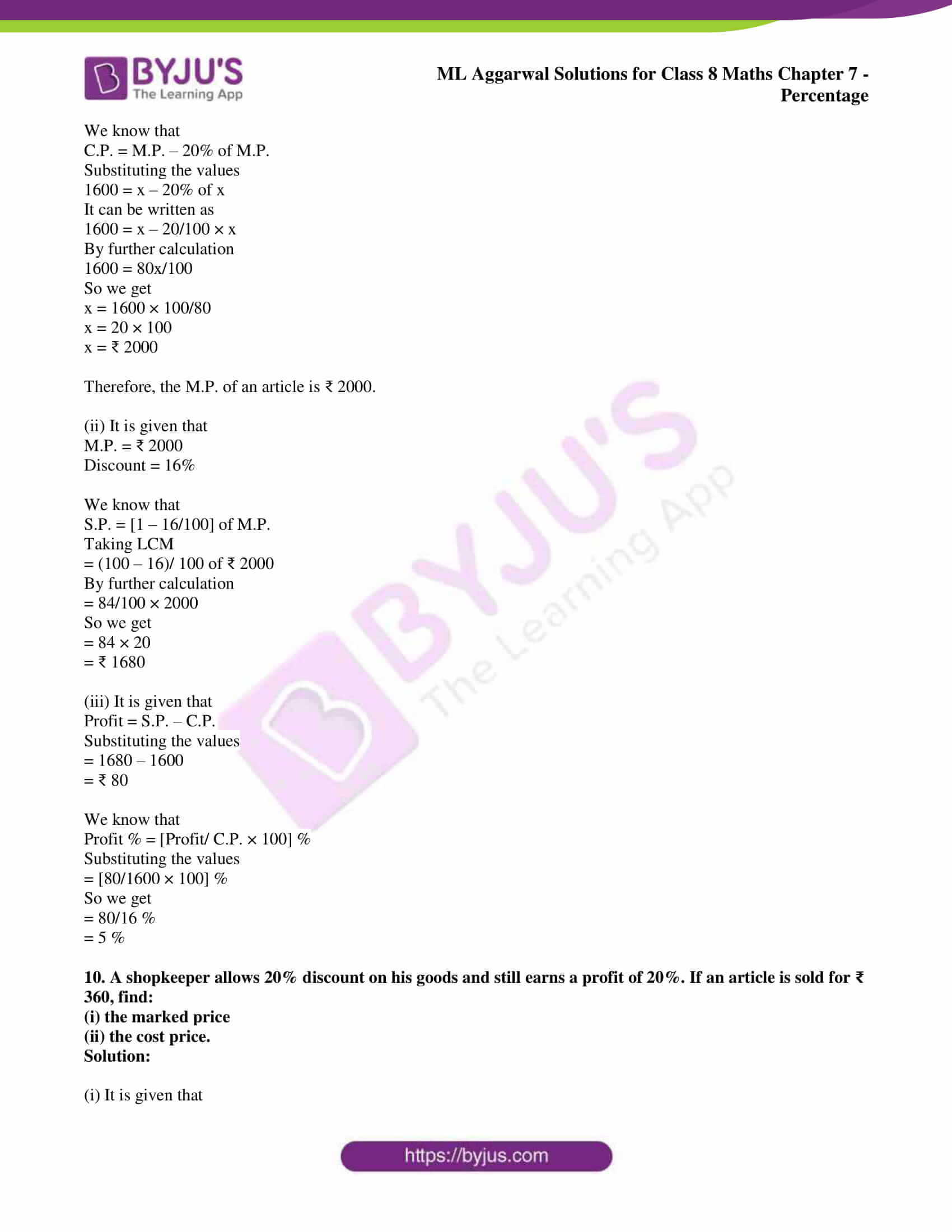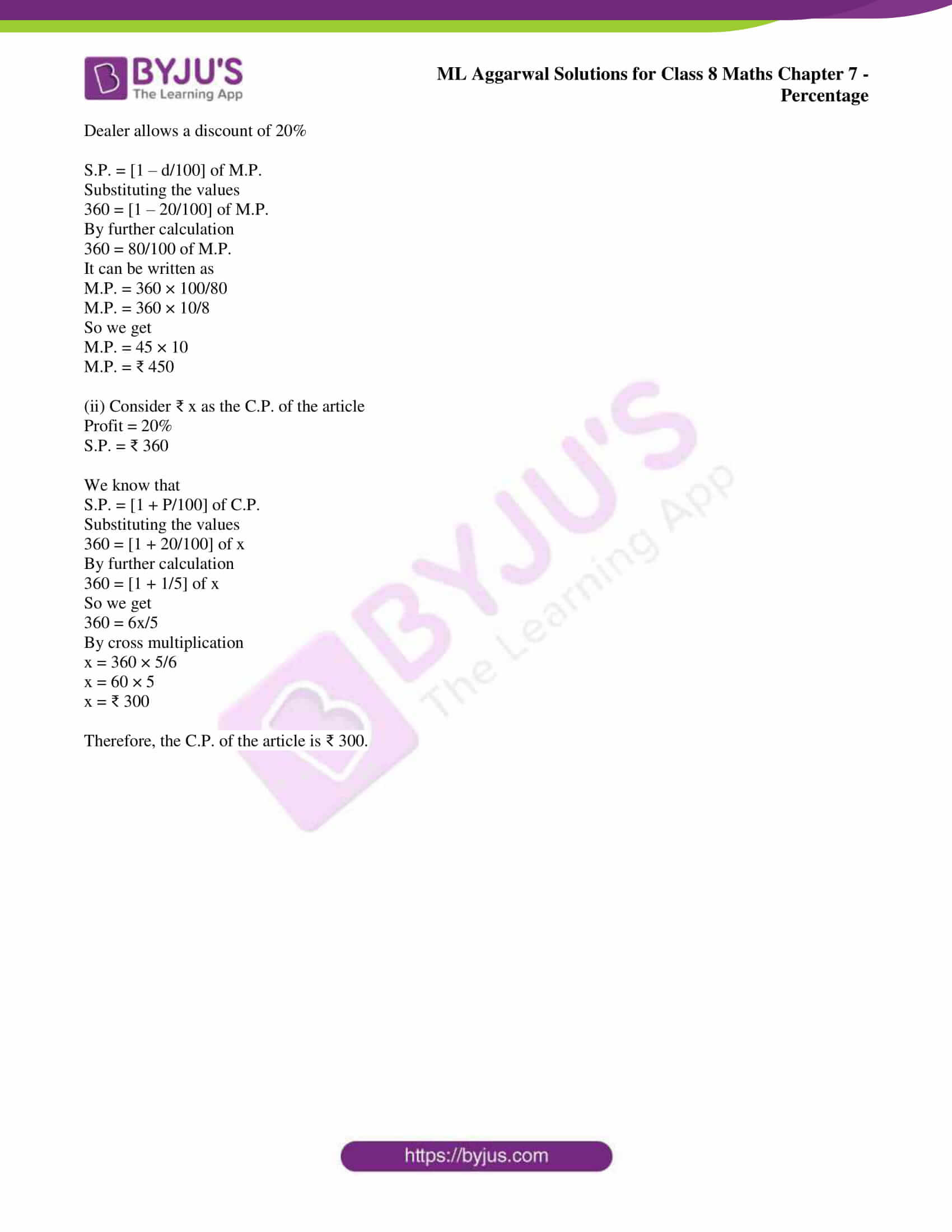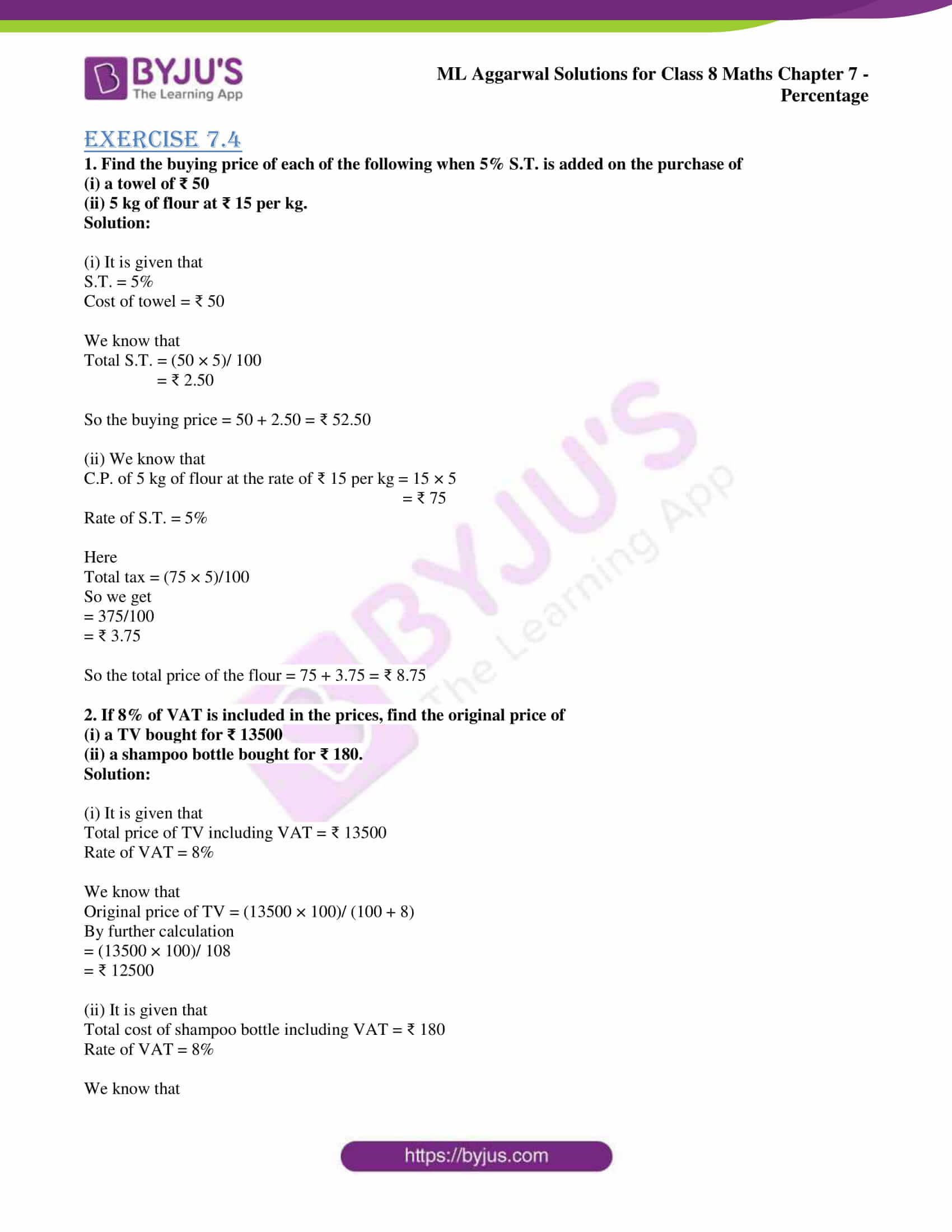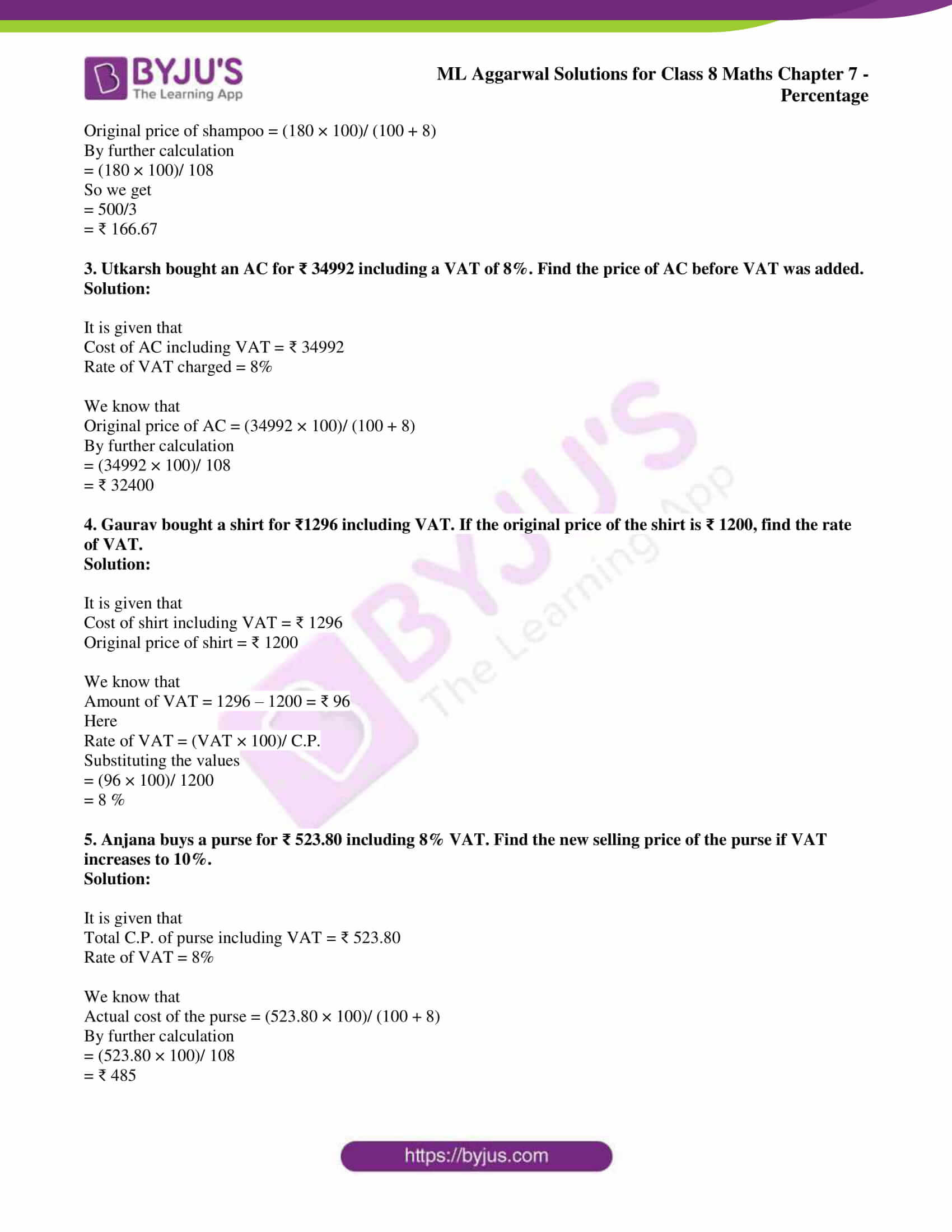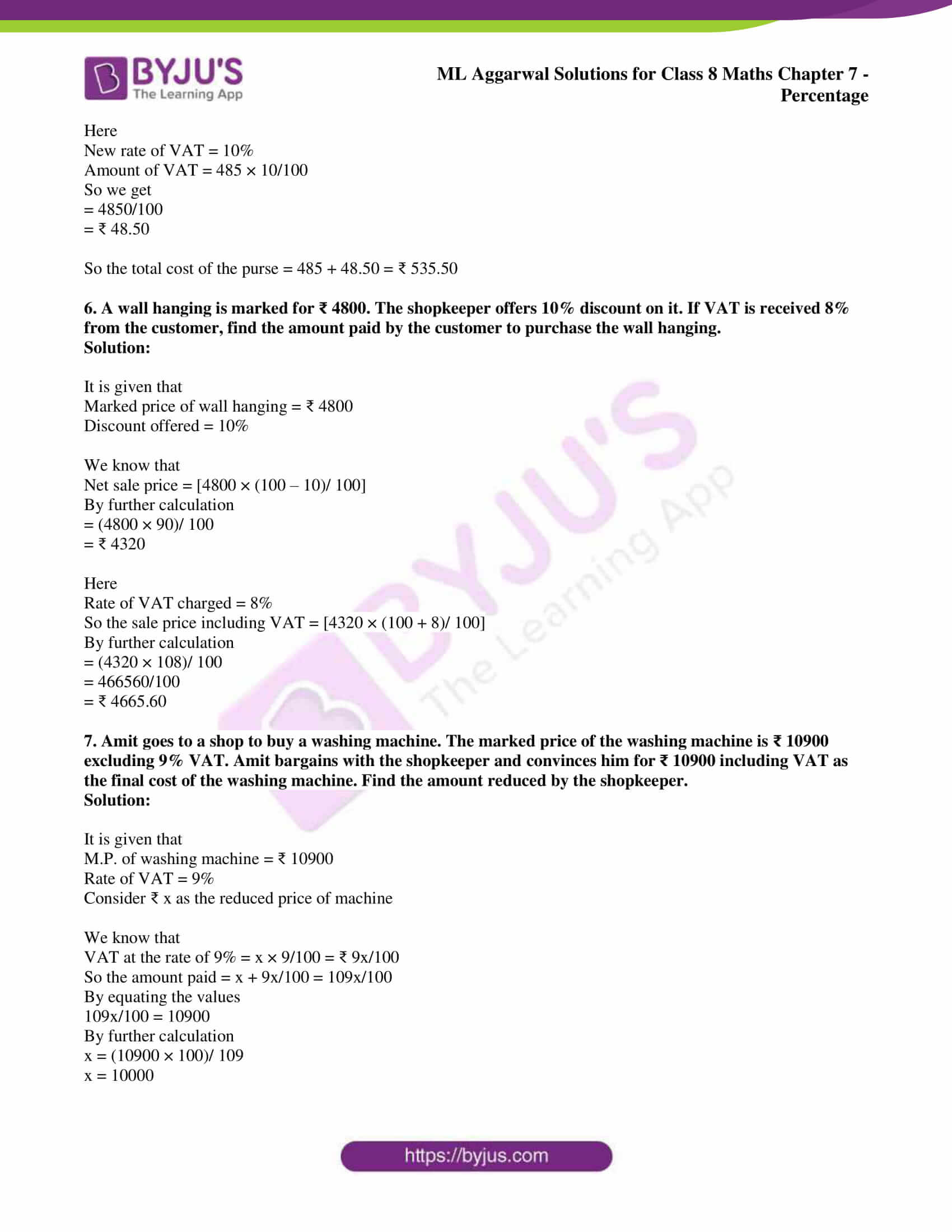## Access ML Aggarwal Solutions for Class 8 Maths Chapter 7: Percentage

Exercise 7.1

1. Express the following percentages as fractions:

(i) 356%

(ii) 2 ½%

(iii) 16 2/2 %

Solution:

(i) 356%

It can be written as

= 356/100

By further simplification

= 89/25

= 3 14/25

(ii) 2 ½%

It can be written as

= 5/2%

By further calculation

= 5/ (2 × 100)

= 1/40

(iii) 16 2/3 %

It can be written as

= 50/3 %

By further calculation

= 50/3 × 1/100

= 1/6

2. Express the following fractions as percentages:

(i) 3/2

(ii) 9/20

(iii) 1 ¼

Solution:

(i) 3/2

It can be written as

= 3/2 × 100%

= 150%

(ii) 9/20

It can be written as

= 9/20 × 100%

= 45%

(iii) 1 ¼

It can be written as

= 5/4 × 100%

= 125%

3. Express the following fractions as decimals. Then express the decimals as percentages:

(i) ¾

(ii) 5/8

(iii) 3/16

Solution:

(i) ¾ = 0.75

It can be written as

¾ = ¾ × 100%

By further calculation

= 3 × 25%

= 75%

(ii) 5/8 = 0.625

It can be written as

5/8 = 5/8 × 100%

By further calculation

= 5/2 × 25%

= 125/2%

= 62.5%

(iii) 3/16 = 0.1875

It can be written as

3/16 = 3/16 × 100%

By further calculation

= ¾ × 25%

= 75/4%

= 18.75%

4. Express the following fractions as decimals correct to four decimal places. Then express the decimals as percentages:

(i) 2/3

(ii) 5/6

(iii) 4/7

Solution:

(i) 2/3 = 0.6667

By correcting to four decimal places

2/3 = 0.6667 × 100% = 66.67%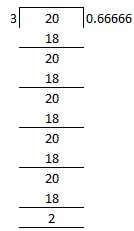(ii) 5/6 = 0.8333

By correcting to four decimal places

5/6 = 0.8333 × 100% = 83.33%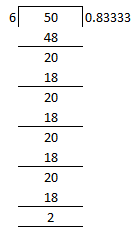(iii) 4/7 = 0.5714

By correcting to four decimal places

4/7 = 0.5714 × 100% = 57.14%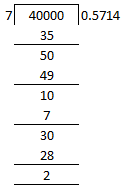5. Express the following ratios as percentages:

(i) 17: 20

(ii) 13: 18

(iii) 93: 80

Solution:

(i) 17: 20

It can be written as

17: 20 = 17/ 20

By further calculation

= 17/20 × 100%

So we get

= 17 × 5%

= 85%

(ii) 13: 18

It can be written as

13: 18 = 13/18

By further calculation

= 13/18 × 100%

So we get

= 13/9 × 50%

= 650/9 %

= 72 2/9%

(iii) 93: 80

It can be written as

93: 80 = 93/80

By further calculation

= 93/80 × 100%

So we get

= 93/4 × 5%

= 465/4%

= 116.25 %

6. Express the following percentages as decimals:

(i) 20%

(ii) 2%

(iii) 3 ¼ %

Solution:

(i) 20%

It can be written as

= 20/100

So we get

= 0.20

= 0.2

(ii) 2%

It can be written as

= 2/100

So we get

= 0.02

(iii) 3 ¼

It can be written as

= 13/4

Multiply the denominator by 100

= 13/ (4 × 100)

= 13/ 400

By further calculation

= 3.25/100

= 0.325

7. Find the value of:

(i) 27 % of ₹ 50

(ii) 6 ¼ % of 25 kg

Solution:

(i) 27 % of ₹ 50

It can be written as

= 27/100 of ₹50

By further calculation

= 27/100 × 50

So we get

= 27/2

= ₹ 13.50

(ii) 6 ¼ % of 25 kg

It can be written as

= 25/4% of 25 kg

By further calculation

= 25/ (4 × 100) of 25 kg

= (25 × 25)/ (4 × 100)

So we get

= 25/16

= 1 9/16 kg

8. What percent is:

(i) 300 g of 2 kg

(ii) ₹ 7.50 of ₹ 6

Solution:

(i) Required percentage = [300 gram/ 2 kg × 100] %

It can be written as

= [300 gram/ (2 × 1000 gram) × 100] %

By further calculation

= [300/ (2 × 1000) × 100] %

So we get

= (30/2) %

= 15 %

(ii) Required percentage = [₹ 7.50/ ₹ 6 × 100] %

It can be written as

= [7.50/ 6 × 100] %

By further calculation

= [7.50/3 × 50] %

So we get

= [2.50 × 50] %

= 125%

9. What percent of:

(i) 50 kg is 65 kg

(ii) ₹ 9 is ₹ 4

Solution:

(i) Consider x% of 50 kg as 65 kg

x% of 50 kg = 65 kg

It can be written as

x/ 100 × 50 = 65

By further calculation

x/ 2 = 65

By cross multiplication

x = 130

Therefore 130% of 50 kg is 65 kg.

(ii) Consider x% of ₹ 9 is ₹ 4

x% of ₹ 9 = ₹ 4

It can be written as

x/ 100 × 9 = 4

By further calculation

x = 4 × 100/9

So we get

x = 400/9

x = 44 4/9

Therefore, 44 4/9 % of ₹ 9 is ₹ 4.

10. (i) If 16 2/3 % of a number is 25, find the number.

(ii) If 13.25 % of a number is 159, find the number.

Solution:

(i) Consider the number as x

16 2/3 % of x = 25

By further calculation

50/3 % of x = 25

It can be written as

50/3 × 1/100 of x = 25

So we get

x = (25 × 3 × 100)/ 50

x = 150

Therefore, the number is 150.

(ii) Consider the number as x

13.25% of x = 159

It can be written as

13.25/ 100 of x = 159

By further calculation

x = (159 × 100)/ 13.25

Multiply and divide by 100

x = (159 × 100 × 100)/ 1325

So we get

x = (159 × 4 × 100)/ 53

x = 3 × 4 × 100

x = 1200

Therefore, the number is 1200.

11. (i) Increase the number 60 by 30 %

(ii) Decrease the number 750 by 10%

Solution:

(i) New number = (1 + 30/100) of 60

By further calculation

= (1 + 3/10) × 60

So we get

= 13/10 × 60

= 78

(ii) New number = (1 – 10/100) of 750

By further calculation

= (1 – 1/10) × 750

So we get

= 9/10 × 750

= 9 × 75

= 675

12. (i) What number when increased by 15% becomes 299?

(ii) On decreasing the number by 18%, it becomes 697. Find the number.

Solution:

(i) Consider the original number as x

Here

New number = (1 + 15/100) of original number

Substituting the values

299 = (1 + 3/20) × x

Taking LCM

299 = [(20 + 3)/ 20] × x

By further calculation

299 = 23/20 × x

So we get

x = (299 × 20)/ 23

x = 13 × 20

x = 260

Therefore, the original number is 260.

(ii) Consider the original number as x

Here

New number = (1 – 18/100) of original number

Substituting the values

697 = (1 – 18/100) of x

Taking LCM

697 = [(100 – 18)/ 100] × x

By further calculation

697 = 82/100 × x

So we get

x = (697 × 100)/ 82

x = (697 × 50)/ 41

By further simplification

x = 17 × 50

x = 850

Therefore, the original number is 850.

13. Mr. Khanna spent 83% of his salary and saved ₹ 1870. Calculate his monthly salary.

Solution:

It is given that

Mr. Khanna spent 83% of his salary

Savings = 100 – 83 = 17%

So 17% of his salary = ₹ 1870

We know that

His salary = ₹ (1870 × 100)/ 17

= ₹ 11000

14. In school, 38% of the students are girls. If the number of boys is 1023, find the total strength of the school.

Solution:

It is given that

No. of girls in school = 38%

No. of boys in school = (100 – 38) % = 62%

Consider x as the total strength of school

62% of x = 1023

It can be written as

62/100 × x = 1023

By further calculation

x = 1023 × 100/62

So we get

x = 1023 × 50/31

x = 33 × 50

x = 1650

Therefore, the total strength of the school is 1650.

15. The price of an article increases from ₹ 960 to ₹ 1080. Find the percentage increase in the price.

Solution:

It is given that

Increase in the price of an article = 1080 – 960 = ₹ 120

We know that

Percentage increase in the price = 120/960 × 100%

By further calculation

= 1/8 × 100%

So we get

= 100/8 %

= 25/2 %

= 12.5 %

16. In a straight contest, the loser polled 42% votes and lost by 14400 votes. Find the total number of votes polled. If the total number of eligible voters was 1 lakh, find what percentage of voters did not vote.

Solution:

It is given that

Losing candidate got 42% of the votes polled

Votes secured by winning candidate = (100 – 42) % of the votes polled

= 58 % of the votes polled

So the difference of votes = 58% – 42%

= 16% of the votes polled

We know that

16% of the votes polled = 14400

16%/100 of the votes polled = 14400

So the votes polled = 14400 × 100/16

By further calculation

= 900 × 100

= 90000

Here

Total number of eligible voters = 100000

No. of voters who did not vote = 100000 – 90000

= 10000

Percentage of voters did not vote = [10000/ 100000 × 100] %

By further calculation

= 10000/1000 %

= 10 %

17. Out of 8000 candidates, 60% were boys. If 80% of the boys and 90% of the girls passed the exam, find the number of candidates who failed.

Solution:

It is given that

Total number of candidates = 8000

No. of boys = 60% of 8000

By further calculation

= 60/100 × 8000

So we get

= 60 × 80

= 4800

No. of girls = 8000 – 4800 = 3200

No. of passed boys = 80% of No. of boys

It can be written as

= 80/100 × 4800

So we get

= 80 × 48

= 3840

No. of passed girls = 90% of No. of girls

It can be written as

= 90/100 × 3200

So we get

= 90 × 32

= 2880

No. of passed candidates = 3840 + 2880 = 6720

No. of failed candidates = 8000 – 6720 = 1280

Therefore, the number of candidates who failed is 1280.

18. In an exam, ¼ of the students failed both in English and Maths, 35% of the students failed in Maths and 30% failed in English.

(i) Find the percentage of students who failed in any of the subjects.

(ii) Find the percentage of students who passed in both subjects.

(iii) If the number of students who failed only in English was 25, find the total number of students.

Solution:

Consider the total number of students = x

No. of students who failed both in English and Maths = ¼ of x = x/4

No. of students who failed in Maths = 35% of x

It can be written as

= 35/100 of x

By further calculation

= 7/20 × x

= 7x/20

No. of students who failed in English = 30% of x

It can be written as

= 30/100 × x

By further calculation

= 3/10 × x

= 3x/10

(i) No. of students who failed in any of the subject = (7x/20 + 3x/10) – x/4

Taking LCM

= (7x + 6x)/ 20 – x/4

So we get

= (13x – 5x)/ 20

= 8x/20

Percentage of students who failed in any of the subject = 8x/20/x × 100%

We can write it as

= 8x/20 × 1/x × 100%

By further calculation

= 8 × 1 × 5%

= 40%

(ii) Percentage of students who passed in both the subjects = 100 – 40 = 60%

(iii) It is given that

No. of students who failed only in English = 25

We can write it as

3x/10 – x/4 = 25

No. of students who failed only in English = 3x/10 – x/4

Taking LCM

(6x – 5x)/ 20 = 25

By further calculation

x = 25 × 20

x = 500

Therefore, the total number of students is 500.

19. On increasing the price of an article by 16%, it becomes ₹ 1479. What was its original price?

Solution:

Consider the original price of an article = ₹ x

1479 = (1 + 16/100) of original price

It can be written as

1479 = [(100 + 16)/ 100] × ₹ x

By further calculation

1479 = 116/100 × x

So we get

116x/100 = 1479

By separating the terms

x = (1479 × 100)/ 116

x = (1479 × 25)/ 29

By division

x = 51 × 25

x = 1275

Therefore, the original price of an article is ₹ 1275.

20. Pratibha reduced her weight by 15%. If now she weighs 59.5 kg, what was her earlier weight?

Solution:

It is given that

Weight reduced by Pratibha = 15%

Present weight of Pratibha = 59.5 kg

Consider her original weight = 100

Reduced weight = 100 – 15 = 85%

Here

85% of her original weight = 59.5 kg

So her original weight = (59.5 × 100)/ 85

By further calculation

= 0.7 × 100

= 70 kg

21. In a sale, a shop reduces all its prices by 15%. Calculate:

(i) the cost of an article which was originally priced at ₹ 40.

(ii) the original price of an article which was sold for ₹ 20.40.

Solution:

It is given that

Rate of reduction = 15%

(i) Original price of an article = ₹ 40

Rate of reduction = 15%

By further calculation

Reduction = (40 × 15)/ 100 = ₹ 6

So the sale price = 40 – 6 = ₹ 34

(ii) Sale price = ₹ 20.40

Rate of reduction = 15%

We know that

Cost price = (SP × 100)/ (100 – reduction %)

Substituting the values

= (20.40 × 100)/ (100 – 15)

By further calculation

= (2040 × 100)/ (100 × 85)

= ₹ 24

22. Increase the price of ₹ 200 by 10% and then decrease the new price by 10%. Is the final price same as the original one?

Solution:

It is given that

Rate of increase = 10%

Rate of decrease = 10%

Price of article = ₹ 200

Here

Increased price = ₹ 200 × (100 + 10)/ 100

By further calculation

= ₹ 200 × 110/100

= ₹ 220

We know that

Decreased price = ₹ 200 × (100 – 10)/ 100

So we get

= ₹ 220 × 90/100

= ₹ 198

No, the final price is not as same as the original one.

23. Chandani purchased some parrots. 20% flew away and 5% died. Of the remaining, 45% were sold. Now 33 parrots remain. How many parrots had Chandani purchased?

Solution:

Consider Chandani purchased x parrots

No. of parrots flew away = 20% of x

It can be written as

= 20/100 × x

So we get

= 1/5 × x

= x/5

No. of parrots died = 5% of x

It can be written as

= 5/100 × x

= x/20

No. of parrots remaining = x – (x/5 + x/20)

Taking LCM

= x – [(4x + x)/ 20]

By further calculation

= x – 5x/20

= x – x/4

Taking LCM

= (4x – x)/ 4

= 3x/4

No. of sold parrots = 45% of 3x/4

It can be written as

= 45/100 × 3x/4

By further calculation

= 9/20 × 3x/4

= 27x/80

No. of parrots which are not sold = 3x/4 – 27/80

Taking LCM

= (60x – 27x)/ 80

= 33x/80

Based on the question

33x/80 = 33

By cross multiplication

33x = 33 × 80

So we get

x = (33 × 80)/ 33

x = 80

Therefore, Chandani purchased 80 parrots.

24. A candidate who gets 36% marks in an examination fails by 24 marks but another candidate, who gets 43% marks, gets 18 more marks than the minimum pass marks. Find the maximum marks and the percentage of pass marks.

Solution:

Consider x as the maximum marks

Marks secured by the first candidate = 36% of x

It can be written as

= 36/100 × x

= 36x/ 100

Marks secured by another candidate = 43% of x

It can be written as

= 43/100 × x

= 43x/ 100

The qualifying marks are same for both the candidates

So according to the question

36x/100 + 24 = 43x/100 – 18

By further calculation

24 + 18 = 43x/100 – 36x/ 100

Taking LCM

42 = (43x – 36x)/ 100

42 = 7x/100

By cross multiplication

x = 42 × 100/7

x = 6 × 100

x = 600

Here the maximum marks = 600

Marks secured by first candidate = 36/100 × 600 = 36 × 6 = 216

Qualifying marks = 216 + 24 = 240

So the percentage of qualifying marks = (240/600 × 100) %

By further calculation

= 240/6 %

= 40 %

Hence, the maximum mark is 600 and the percentage of pass marks is 40%.

Exercise 7.2

1. Find the profit or loss percentage, when:

(i) C.P. = ₹ 400, S.P. = ₹ 468

(ii) C.P. = ₹ 13600, S.P. = ₹ 12104

Solution:

(i) It is given that

C.P. = ₹ 400, S.P. = ₹ 468

Profit = S.P. – C.P.

Substituting the values

= 468 – 400

= ₹ 68

Here

Profit % = (Profit × 100)/ C.P.

Substituting the values

= (68 × 100)/ 400

= 17 %

(ii) It is given that

C.P. = ₹ 13600, S.P. = ₹ 12104

Loss = C.P. – S.P.

Substituting the values

= 13600 – 12104

= ₹ 1496

Here

Loss % = [Loss/C.P. × 100] %

Substituting the values

= [1496/ 13600 × 100] %

So we get

= 1496/ 136 %

= 11 %

2. By selling an article for ₹ 1636.25, a dealer gains ₹ 96.25. Find his gain per cent.

Solution:

It is given that

S.P. of an article = ₹ 1636.25

Gain = ₹ 96.25

So the C.P. = S.P. – Gain

Substituting the values

= 1636.25 – 96.25

= ₹ 1540

We know that

Gain % = [Gain/ C.P. × 100] %

Substituting the values

= [96.25/1540 × 100] %

By further calculation

= 9625/1540 %

= 1925/308 %

So we get

= 25/4 %

= 6 ¼ %

3. By selling an article for ₹ 770, a man incurs a loss of ₹ 110. Find his loss percentage.

Solution:

It is given that

S.P. of an article = ₹ 770

Loss = ₹ 110

We know that

C.P. = S.P. + Loss

Substituting the values

= 770 + 110

= ₹ 880

Here

Loss % = [Loss/ C.P. × 100] %

Substituting the values

= [110/ 880 × 100] %

By further calculation

= 100/8 %

So we get

= 25/2 %

= 12.5 %

4. Rashida bought 25 dozen eggs at the rate of ₹ 9.60 per dozen. 30 eggs were broken in the transaction and she sold the remaining eggs at one rupee each. Find her gain or loss percentage.

Solution:

It is given that

C.P. of one dozen eggs = ₹ 9.60

C.P. of 25 dozen eggs = 25 × 9.60 = ₹ 240

No. of eggs = 25 dozen = 25 × 12 = 300

No. of eggs broken in transaction = 30

No. of remaining eggs = 300 – 30 = 270

We know that

S.P. of one egg = ₹ 1

S.P. of 270 eggs = 1 × 270 = ₹ 270

So the profit = S.P. – C.P.

Substituting the values

= 270 – 240

= ₹ 30

Here

Profit % = [Profit/ C.P. × 100] %

Substituting the values

= [30/240 × 100] %

So we get

= 100/8 %

= 25/2 %

= 12.5 %

5. The cost of an article was ₹ 20000 and ₹ 1400 were spent on its repairs. If it is sold for a profit of 20 %, find the selling price of the article.

Solution:

It is given that

Cost of an article = ₹ 20000

Cost of its repair = ₹ 1400

So the total cost = 20000 + 1400 = ₹ 21400

Profit = 20 %

We know that

S.P. = [C.P. × (100 + Profit %)]/ 100

Substituting the values

= [21400 × (100 + 20)]/ 100

By further calculation

= (21400 × 120)/ 100

= ₹ 25680

6. A shopkeeper buys 200 bicycles at ₹ 1200 per bicycle. He spends ₹ 30 per bicycle on transportation. He also spends ₹ 4000 on advertising. Then he sells all the bicycles at ₹ 1350 per piece. Find his profit or loss. Also, calculate it as a percentage.

Solution:

It is given that

C.P. of one bicycle = ₹ 1200

C.P. of 200 bicycle = 1200 × 200 = ₹ 240000

Expenditure on transportation for one bicycle = ₹ 30

Expenditure on transportation for 200 bicycle = 30 × 200 = ₹ 6000

Expenditure on advertising = ₹ 4000

We know that

Net C.P. of the bicycle = 240000 + 6000 + 4000

= ₹ 250000

S.P. of 200 bicycle at ₹ 1350 per bicycle = 200 × 1350

= ₹ 270000

So profit = S.P – C.P.

Substituting the values

= 270000 – 250000

= ₹ 20000

Here

Profit % = [Profit/ C.P. × 100] %

Substituting the values

= [20000/ 250000 × 100] %

So we get

= 200/25 %

= 8%

7. The cost price of an article is 90% of its selling price. Find his profit percentage.

Solution:

Consider ₹ x as the S.P. of an article

C.P. of an article = 90% of ₹ x

It can be written as

= 90/100 × ₹ x

= ₹ 9x/10

We know that

Profit = S.P. – C.P.

Substituting the values

= x – 9x/10

Taking LCM

= (10x – 9x)/ 10

= ₹ x/10

Here

Profit % = [Profit/ C.P. × 100] %

Substituting the values

= [x/10/ 9x/10 × 100] %

It can be written as

= [x/10 × 10/9x × 100] %

So we get

= 100/ 9 %

= 11 1/9 %

8. Rao bought notebooks at the rate of 4 for ₹ 35 and sold them at the rate of 5 for ₹ 58. Calculate

(i) his gain percentage.

(ii) the number of notebooks he should sell to earn a profit of ₹ 171.

Solution:

Consider the number of note books bought = 20

Here the LCM of 4 and 5 is 20

C.P. of the note books = 35/4 × 20

= 35 × 5

= ₹ 175

S.P. of the note books = 58/5 × 20

= 58 × 4

= ₹ 232

(i) We know that

Gain = S.P. – C.P.

Substituting the values

= 232 – 175

= ₹ 57

Here

Gain % = [Gain/ C.P. × 100] %

Substituting the values

= [57/ 175 × 100] %

By further calculation

= [57/7 × 4] %

So we get

= 228/7 %

= 32 4/7 %

(ii) When the profit is ₹ 57, the number of note books sold = 20

When the profit is ₹ 1, the number of note books sold = 20/57

When the profit is ₹ 171, the number of note books sold = 20/57 × 171

= 20 × 3

= 60

9. A vendor buys bananas at 3 for a rupee and sells at 4 for a rupee. Find his profit or loss percentage.

Solution:

Consider the number of bananas bought = 12

Here LCM f 3 and 4 is 12

We know that

C.P. of bananas = 1/3 × 12 = ₹ 4

S.P. of bananas = ¼ × 12 = ₹ 3

Here

Loss = C.P. – S.P.

Substituting the values

= 4 – 3

= ₹ 1

Loss % = [Loss/ C.P. × 100] %

Substituting the values

= [1/4 × 100] %

So we get

= 100/4 %

= 25 %

10. A shopkeeper buys a certain number of pens. If the selling price of 5 pens is equal to the cost price of 7 pens, find his profit or loss percentage.

Solution:

Consider ₹ x as the C.P. of 7 pens

C.P. of 1 pen = ₹ x/7

Based on the question

S.P. of 5 pens = ₹ x

S.P. of 1 pen = ₹ x/5

Profit = S.P. – C.P.

Substituting the values

= x/7 – x/5

Taking LCM

= (7x – 5x)/ 35

= ₹ 2x/ 35

We know that

Profit % = Profit/C.P. × 100 %

Substituting the values

= 2x/ 35/ x/7 × 100 %

It can be written as

= 2x/35 × 7/x × 100 %

By further calculation

= 2/5 × 100 %

So we get

= 2 × 20 %

= 40 %

11. Find the selling price, when:

(i) Cost price = ₹ 2360, Profit = 8 %

(ii) Cost price = ₹ 380, Loss = 7.5 %

Solution:

(i) It is given that

Cost price = ₹ 2360, Profit = 8%

We know that

S.P. = (100 + Profit %)/ 100 × C.P.

Substituting the values

= (100 + 8)/ 100 × 2360

By further calculation

= 108/ 100 × 2360

So we get

= 108/ 10 × 236

= ₹ 2548.80

(ii) It is given that

Cost price = ₹ 380, Loss = 7.5 %

We know that

S.P. = (100 – Loss %)/ 100 × C.P.

Substituting the values

= (100 – 7.5)/ 100 × 380

By further calculation

= 92.5/ 100 × 380

So we get

= 9.25 × 38

= ₹ 351.50

12. A dealer bought a number of eggs at ₹ 18 a dozen and sold them at 50% profit. Find the selling price per egg.

Solution:

It is given that

C.P. of one dozen eggs = 12 eggs = ₹ 18

Profit = 15%

We know that

S.P. of 12 eggs = [1 + 50/ 100] of ₹ 18

It can be written as

= (150/100 × 18)

By further calculation

= (3/2 × 18)

So we get

= 3 × 9

= ₹ 27

S.P. of 1 egg = ₹ 27/12

So we get

= ₹ 9/4

= ₹ 2.25

Exercise 7.3

1. Find the discount and the selling price, when:

(i) the marked price = ₹ 575, discount = 12%

(ii) the printed price = ₹ 12750, discount = 8 1/3%

Solution:

(i) the marked price = ₹ 575, discount = 12%

Here

Amount of discount = 12 % of ₹ 575

It can be written as

= (12/100 × 575)

By further calculation

= (12/4 × 23)

So we get

= 3 × 23

= ₹ 69

We know that

Net sale price = M.P. – discount

Substituting the values

= 575 – 69

= ₹ 506

(ii) the printed price = ₹ 12750, discount = 8 1/3% = 25/3 %

Here

Amount of discount = 25/3 % of ₹ 12750

It can be written as

= [25/ (3 × 100) × 12750]

By further calculation

= (25/30 × 1275)

So we get

= (5/6 × 1275)

= ₹ 1062.50

We know that

Net sale price = M.P. – discount

Substituting the values

= 12750 – 1062.50

= ₹ 11687.50

2. Find the discount and the discount percentage, when:

(i) marked price = ₹ 780, selling price = ₹ 721.50

(ii) advertised price = ₹ 28500, selling price = ₹ 24510

Solution:

(i) marked price = ₹ 780, selling price = ₹ 721.50

We know that

Discount = M.P. – Selling price

Substituting the values

= 780 – 721.50

= ₹ 58.50

Here

Discount % = [Discount/M.P. × 100] %

Substituting the values

= [58.50/780 × 100] %

By further calculation

= 5850/780 %

So we get

= 585/78 %

= 7.5 %

(ii) advertised price = ₹ 28500, selling price = ₹ 24510

We know that

Discount = Advertised price – Selling Price

Substituting the values

= 28500 – 24510

= ₹ 3990

Here

Discount % = [Discount/ advertised price × 100] %

Substituting the values

= [3990/ 28500 × 100] %

So we get

= 3990/ 285 %

= 14 %

3. A notebook is marks at ₹ 30. Find the price a student pays for a dozen notebooks if he gets 15% discount.

Solution:

It is given that

M.P. of one notebook = ₹ 30

M.P. of one dozen notebooks = 30 × 12 = ₹ 360

Discount = 15%

We know that

Amount of discount = 15% of M.P.

It can be written as

= 15% of ₹ 360

By further calculation

= (15/100 × 360)

So we get

= (15/10 × 36)

= (3/2 × 36)

On further simplification

= 3 × 18

= ₹ 54

Price a student pays for a dozen notebooks = 360 – 54 = ₹ 306

4. A dealer gave 9% discount on an electric fan and charges ₹ 728 from the customer. Find the marked price of the fan.

Solution:

Consider ₹ x as the M.P. of the fan

Discount = 9%

We know that

Amount of discount = 9% of ₹ x

It can be written as

= 9/100 × x

= ₹ 9x/100

Here

Charges for customer = ₹ x – ₹9x/100

Substituting the values

728 = (100x – 9x)/ 100

By further calculation

728 = 91x/100

So we get

x = (728 × 100)/ 91

x = 8 × 100

x = 800

Therefore, the marked price of the fan is ₹ 800.

5. The list price of an article is ₹ 800 and a dealer is selling it at a discount of 20 %. Find:

(i) the selling price of the article.

(ii) the cost price of the article if he makes 25% profit on selling it.

Solution:

(i) It is given that

M.P. = ₹ 800

Discount = 20%

We know that

S.P. = [1 – d/100] of M.P.

Substituting the values

S.P. = [1 – 20/100] of ₹ 800

By further calculation

S.P. = 80/100 × 800

S.P. = ₹ 640

Therefore, the selling price is ₹ 640.

(ii) It is given that

S.P. = ₹ 640

Profit = 25%

We know that

S.P. = [1 + P/100] of C.P.

Substituting the values

640 = [1 + 25/100] of C.P.

By further calculation

640 = 125/100 of C.P.

So we get

C.P. = [640 × 100/125]

C.P. = 128 × 4

C.P. = ₹ 512

6. A shopkeeper marks his goods at such a price that would give him a profit of 10% after allowing a discount of 12%. If an article is marked at ₹ 2250, find its:

(i) selling price

(ii) cost price.

Solution:

(i) It is given that

M.P. of an article = ₹ 2250

Discount = 12 %

We know that

S.P. = [1 – d/100] of M.P.

Substituting the values

S.P. = [1 – 12/100] of ₹ 2250

By taking LCM

S.P. = (100 – 12)/100 × 2250

By further calculation

S.P. = 88/100 × 2250

So we get

S.P. = 88/4 × 90

S.P. = 22 × 90

S.P. = ₹ 1980

(ii) It is given that

S.P. = ₹ 1980

Profit = 10%

We know that

S.P. = [1 + P/100] of C.P.

Substituting the values

1980 = [1 + 10/100] of C.P.

By further calculation

1980 = 110/100 of C.P.

So we get

C.P. = 1980 × 100/100

C.P. = 18 × 100

C.P. = ₹ 1800

Therefore, the cost price is ₹ 1800.

7. A shopkeeper purchased a calculator for ₹ 650. He sells it at a discount of 20% and still makes a profit of 20%. Find:

(i) the selling price

(ii) marked price

Solution:

(i) It is given that

C.P. = ₹ 650

Profit = 20%

We know that

S.P. = [1 + P/100] of C.P.

Substituting the values

= [1 + 20/100] × 650

By further calculation

= 120/100 × 650

So we get

= 12 × 65

= ₹ 780

Therefore, the selling price of the calculator is ₹ 780.

(ii) It is given that

S.P. = ₹ 780

Discount = 20%

We know that

S.P. = [1 – d/100] of M.P

Substituting the values

780 = [1 – 20/100] of M.P.

By further calculation

780 = 80/100 of M.P.

It can be written as

M.P. = 780 × 100/80

So we get

M.P. = 780 × 10/8

M.P. = 7800/8

M.P. = ₹ 975

Therefore, the marked price of the calculator is ₹ 975.

8. A shopkeeper buys a dinner set for ₹ 1200 and marks it 80% above the cost price. If he gives 15 % discount on it, find:

(i) the marked price

(ii) the selling price

(iii) his profit percentage.

Solution:

(i) It is given that

C.P. of a dinner set = ₹ 1200

We know that

M.P. = 1200 + 80% of ₹ 1200

By further calculation

= 1200 + 80/100 × 1200

So we get

= 1200 + 80 × 12

By multiplication

= 1200 + 960

= ₹ 2160

(ii) It is given that

M.P. = ₹ 2160

Discount = 15%

We know that

S.P. = (1 – d/100) of M.P.

Substituting the values

= (1 – 15/100) × 2160

By further calculation

= 85/100 × 2160

So we get

= 17/20 × 2160

= 17 × 108

= ₹ 1836

(iii) We know that

Profit = S.P. – C.P.

Substituting the values

= 1836 – 1200

= ₹ 636

Here

Profit % = [Profit/C.P. × 100] %

Substituting the values

= (636/1200 × 100) %

By further calculation

= 636/12 %

= 53 %

9. The cost price of an article is ₹ 1600, which is 20% below the marked price. If the article is sold at a discount of 16%, find:

(i) the marked price

(ii) the selling price

(iii) profit percentage.

Solution:

(i) It is given that

C.P. = ₹ 1600

C.P of an article is 20% below the M.P.

Take ₹ x as the M.P. of an article

We know that

C.P. = M.P. – 20% of M.P.

Substituting the values

1600 = x – 20% of x

It can be written as

1600 = x – 20/100 × x

By further calculation

1600 = 80x/100

So we get

x = 1600 × 100/80

x = 20 × 100

x = ₹ 2000

Therefore, the M.P. of an article is ₹ 2000.

(ii) It is given that

M.P. = ₹ 2000

Discount = 16%

We know that

S.P. = [1 – 16/100] of M.P.

Taking LCM

= (100 – 16)/ 100 of ₹ 2000

By further calculation

= 84/100 × 2000

So we get

= 84 × 20

= ₹ 1680

(iii) It is given that

Profit = S.P. – C.P.

Substituting the values

= 1680 – 1600

= ₹ 80

We know that

Profit % = [Profit/ C.P. × 100] %

Substituting the values

= [80/1600 × 100] %

So we get

= 80/16 %

= 5 %

10. A shopkeeper allows 20% discount on his goods and still earns a profit of 20%. If an article is sold for ₹ 360, find:

(i) the marked price

(ii) the cost price.

Solution:

(i) It is given that

Dealer allows a discount of 20%

S.P. = [1 – d/100] of M.P.

Substituting the values

360 = [1 – 20/100] of M.P.

By further calculation

360 = 80/100 of M.P.

It can be written as

M.P. = 360 × 100/80

M.P. = 360 × 10/8

So we get

M.P. = 45 × 10

M.P. = ₹ 450

(ii) Consider ₹ x as the C.P. of the article

Profit = 20%

S.P. = ₹ 360

We know that

S.P. = [1 + P/100] of C.P.

Substituting the values

360 = [1 + 20/100] of x

By further calculation

360 = [1 + 1/5] of x

So we get

360 = 6x/5

By cross multiplication

x = 360 × 5/6

x = 60 × 5

x = ₹ 300

Therefore, the C.P. of the article is ₹ 300.

Exercise 7.4

1. Find the buying price of each of the following when 5% S.T. is added on the purchase of

(i) a towel of ₹ 50

(ii) 5 kg of flour at ₹ 15 per kg.

Solution:

(i) It is given that

S.T. = 5%

Cost of towel = ₹ 50

We know that

Total S.T. = (50 × 5)/ 100

= ₹ 2.50

So the buying price = 50 + 2.50 = ₹ 52.50

(ii) We know that

C.P. of 5 kg of flour at the rate of ₹ 15 per kg = 15 × 5

= ₹ 75

Rate of S.T. = 5%

Here

Total tax = (75 × 5)/100

So we get

= 375/100

= ₹ 3.75

So the total price of the flour = 75 + 3.75 = ₹ 8.75

2. If 8% of VAT is included in the prices, find the original price of

(i) a TV bought for ₹ 13500

(ii) a shampoo bottle bought for ₹ 180.

Solution:

(i) It is given that

Total price of TV including VAT = ₹ 13500

Rate of VAT = 8%

We know that

Original price of TV = (13500 × 100)/ (100 + 8)

By further calculation

= (13500 × 100)/ 108

= ₹ 12500

(ii) It is given that

Total cost of shampoo bottle including VAT = ₹ 180

Rate of VAT = 8%

We know that

Original price of shampoo = (180 × 100)/ (100 + 8)

By further calculation

= (180 × 100)/ 108

So we get

= 500/3

= ₹ 166.67

3. Utkarsh bought an AC for ₹ 34992 including a VAT of 8%. Find the price of AC before VAT was added.

Solution:

It is given that

Cost of AC including VAT = ₹ 34992

Rate of VAT charged = 8%

We know that

Original price of AC = (34992 × 100)/ (100 + 8)

By further calculation

= (34992 × 100)/ 108

= ₹ 32400

4. Gaurav bought a shirt for ₹1296 including VAT. If the original price of the shirt is ₹ 1200, find the rate of VAT.

Solution:

It is given that

Cost of shirt including VAT = ₹ 1296

Original price of shirt = ₹ 1200

We know that

Amount of VAT = 1296 – 1200 = ₹ 96

Here

Rate of VAT = (VAT × 100)/ C.P.

Substituting the values

= (96 × 100)/ 1200

= 8 %

5. Anjana buys a purse for ₹ 523.80 including 8% VAT. Find the new selling price of the purse if VAT increases to 10%.

Solution:

It is given that

Total C.P. of purse including VAT = ₹ 523.80

Rate of VAT = 8%

We know that

Actual cost of the purse = (523.80 × 100)/ (100 + 8)

By further calculation

= (523.80 × 100)/ 108

= ₹ 485

Here

New rate of VAT = 10%

Amount of VAT = 485 × 10/100

So we get

= 4850/100

= ₹ 48.50

So the total cost of the purse = 485 + 48.50 = ₹ 535.50

6. A wall hanging is marked for ₹ 4800. The shopkeeper offers 10% discount on it. If VAT is received 8% from the customer, find the amount paid by the customer to purchase the wall hanging.

Solution:

It is given that

Marked price of wall hanging = ₹ 4800

Discount offered = 10%

We know that

Net sale price = [4800 × (100 – 10)/ 100]

By further calculation

= (4800 × 90)/ 100

= ₹ 4320

Here

Rate of VAT charged = 8%

So the sale price including VAT = [4320 × (100 + 8)/ 100]

By further calculation

= (4320 × 108)/ 100

= 466560/100

= ₹ 4665.60

7. Amit goes to a shop to buy a washing machine. The marked price of the washing machine is ₹ 10900 excluding 9% VAT. Amit bargains with the shopkeeper and convinces him for ₹ 10900 including VAT as the final cost of the washing machine. Find the amount reduced by the shopkeeper.

Solution:

It is given that

M.P. of washing machine = ₹ 10900

Rate of VAT = 9%

Consider ₹ x as the reduced price of machine

We know that

VAT at the rate of 9% = x × 9/100 = ₹ 9x/100

So the amount paid = x + 9x/100 = 109x/100

By equating the values

109x/100 = 10900

By further calculation

x = (10900 × 100)/ 109

x = 10000

Amount reduced by the shopkeeper = 10900 – 10000

= ₹ 900

Therefore, the amount reduced by the shopkeeper is ₹ 900.